# US5359456A - Objective lens system for endoscopes - Google Patents

Objective lens system for endoscopes Download PDF

## Info

Publication number
US5359456A
US5359456A US07/961,543 US96154392A US5359456A US 5359456 A US5359456 A US 5359456A US 96154392 A US96154392 A US 96154392A US 5359456 A US5359456 A US 5359456A
Authority
US
United States
Prior art keywords
sub
sbsb
refractive index
sup
lens
Prior art date
Legal status (The legal status is an assumption and is not a legal conclusion. Google has not performed a legal analysis and makes no representation as to the accuracy of the status listed.)
Expired - Lifetime
Application number
US07/961,543
Inventor
Akira Kikuchi
Current Assignee (The listed assignees may be inaccurate. Google has not performed a legal analysis and makes no representation or warranty as to the accuracy of the list.)
Olympus Corp
Original Assignee
Olympus Corp
Priority date (The priority date is an assumption and is not a legal conclusion. Google has not performed a legal analysis and makes no representation as to the accuracy of the date listed.)
Filing date
Publication date
Priority to JP29381591A priority Critical patent/JPH05107471A/en
Priority to JP29381191A priority patent/JPH05134171A/en
Priority to JP29392891A priority patent/JPH05127080A/en
Priority to JP3-293928 priority
Priority to JP3-293815 priority
Priority to JP3-293811 priority
Application filed by Olympus Corp filed Critical Olympus Corp
Assigned to OLYMPUS OPTICAL CO., LTD. reassignment OLYMPUS OPTICAL CO., LTD. ASSIGNMENT OF ASSIGNORS INTEREST. Assignors: KIKUCHI, AKIRA
Application granted granted Critical
Publication of US5359456A publication Critical patent/US5359456A/en
Anticipated expiration legal-status Critical
Expired - Lifetime legal-status Critical Current

## Links

• 230000036698 Distribution coefficient Effects 0.000 claims description 19
• 230000003287 optical Effects 0.000 claims description 12
• 230000004075 alteration Effects 0.000 abstract description 88
• 239000000203 mixture Substances 0.000 description 26
• 239000002609 media Substances 0.000 description 19
• 239000000463 material Substances 0.000 description 18
• 239000011521 glass Substances 0.000 description 11
• 206010010071 Coma Diseases 0.000 description 10
• 201000009310 astigmatism Diseases 0.000 description 8
• 239000005304 optical glass Substances 0.000 description 7
• 230000000694 effects Effects 0.000 description 4
• 239000012585 homogenous medium Substances 0.000 description 4
• 230000001629 suppression Effects 0.000 description 2
• 230000001627 detrimental Effects 0.000 description 1
• 230000002708 enhancing Effects 0.000 description 1
• 230000002349 favourable Effects 0.000 description 1
• 238000004519 manufacturing process Methods 0.000 description 1
• 210000000056 organs Anatomy 0.000 description 1
• 239000003973 paint Substances 0.000 description 1
• 238000005728 strengthening Methods 0.000 description 1
• 239000000126 substance Substances 0.000 description 1
• 230000000007 visual effect Effects 0.000 description 1

## Images

•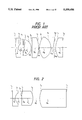•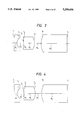•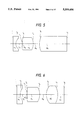•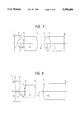•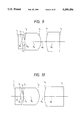•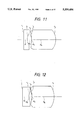•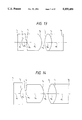•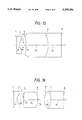•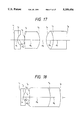•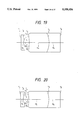•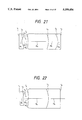•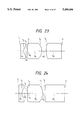•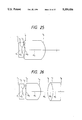•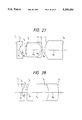•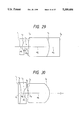•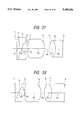•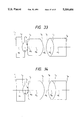•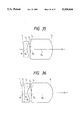•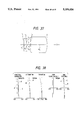•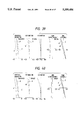•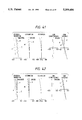•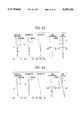•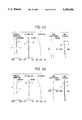•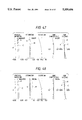•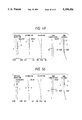•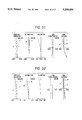•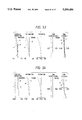•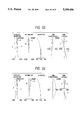•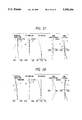•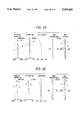•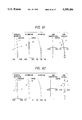•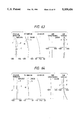•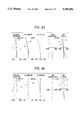•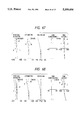•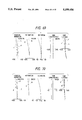••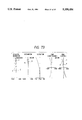## Classifications

• GPHYSICS
• G02OPTICS
• G02BOPTICAL ELEMENTS, SYSTEMS, OR APPARATUS
• G02B9/00Optical objectives characterised both by the number of the components and their arrangements according to their sign, i.e. + or -
• G02B9/12Optical objectives characterised both by the number of the components and their arrangements according to their sign, i.e. + or - having three components only
• GPHYSICS
• G02OPTICS
• G02BOPTICAL ELEMENTS, SYSTEMS, OR APPARATUS
• G02B23/00Telescopes, e.g. binoculars; Periscopes; Instruments for viewing the inside of hollow bodies; Viewfinders; Optical aiming or sighting devices
• G02B23/24Instruments or systems for viewing the inside of hollow bodies, e.g. fibrescopes
• G02B23/2407Optical details
• G02B23/2423Optical details of the distal end
• G02B23/243Objectives for endoscopes
• GPHYSICS
• G02OPTICS
• G02BOPTICAL ELEMENTS, SYSTEMS, OR APPARATUS
• G02B9/00Optical objectives characterised both by the number of the components and their arrangements according to their sign, i.e. + or -
• G02B9/04Optical objectives characterised both by the number of the components and their arrangements according to their sign, i.e. + or - having two components only
• G02B9/10Optical objectives characterised both by the number of the components and their arrangements according to their sign, i.e. + or - having two components only one + and one - component

## Abstract

An objective lens system for endoscopes comprising, in order from the object side, a front lens unit having a negative refractive power, an aperture stop and a rear lens unit having a positive refractive power. This objective lens system is compact, comprises a small number of lens elements and favorably corrected aberrations, especially chromatic aberration, owing to a fact that at least one graded refractive index lens component is used in the front lens unit or the rear lens unit.

## Description

BACKGROUND OF THE INVENTION
a) Field of the Invention
The present invention relates to an objective lens system for endoscopes using a graded refractive index lens component.
b) Description of the Prior Art
There are known many objective lens systems having compositions exemplified by that of the objective lens system disclosed by Japanese Patent Kokai Publication No. Sho 60-46,410 illustrated in FIG. 1. This system is of the retrofocus type which consists of a diverging lens unit (a front lens unit) and a converging lens unit (a rear lens unit) which are disposed before and after respectively an aperture stop.
In the lens system having this composition, the diverging front lens unit has a function to Widen a field angle of the lens system and a role to reduce a Petzval's sum, whereas refractive powers are shared among many lens elements for producing little aberrations in the converging rear lens unit. For this reason, the rear lens unit is composed of three positive lens elements and a cemented lens component. Aberrations are corrected favorably in the objective lens system as a whole by adopting the power distribution described above.
However, this objective lens system comprises a large number of lens elements and has a long total length. Even when the number of lens elements are reduced by using an aspherical surface in this objective lens system, chromatic aberration cannot be corrected favorably though Seidel's aberrations (spherical aberration, astigmatism and coma) and the Petzval's sum can be reduced.
The retrofocus type objective lens system for endoscopes consists of the diverging front lens unit and the converging rear lens unit which are disposed on both sides of the aperture stop as described above, and the front lens unit consists in most cases of a single lens element which has a planar surface on the object side and a concave surface on the image side. The front lens unit has a negative refractive power and, since the lens unit is disposed on the object side of the aperture stop, the front lens unit produces negative lateral chromatic aberration. Since most of the lens elements disposed in the converging rear lens unit have positive refractive powers, the rear lens unit produces remarkable negative lateral chromatic aberration.
In order to correct the remarkable negative lateral chromatic aberration which are produced by the front lens unit and the rear lens unit as described above, it is conventional to use a cemented lens component which consists of a convex lens element made of a glass material having a high dispersing power and a concave lens element made of a glass material having a high dispersing power in the rear lens unit. Further, the lens elements disposed in the converging rear lens unit are made of glass materials which have relatively high refractive indices and relatively weak dispersing powers so that the lens surfaces disposed in the rear lens unit produce lateral chromatic aberration as little as possible.
The objective lens system disclosed by Japanese Patent Kokoku Publication No. Sho 60-46,410 has a wide field angle and faborably corrected aberrations. However, this objective lens system comprises lens elements as many as six, has a complicated composition and requires high cost for manufacturing. This objective lens system has defects that it has a long total length and that, when the objective lens system is used in medical endoscope, it prolongs a hard section of the endoscope which is to be inserted into human bodies, thereby making it impossible to inspect interiors of curved organs.
As a conventional example of an objective lens system for endoscopes using a graded refractive index lens component, there is known the lens system which is disclosed by Japanese Patent Kokoku Publication No. Sho 47-28,061. This lens system has planar surfaces on both sides and uses a graded refractive index lens component which has a refractive index distribution in the radial direction. This objective lens system has a simple composition which is desirable for use with endoscopes, but cannot have a wide field angle which is determined dependently on a distribution of refractive powers therein, has an outside diameter which is large at a certain degree, produces remarkable offaxial aberrations at high image heights, and cannot be used in endoscopes having large outside diameters.
SUMMARY OF THE INVENTION
A primary object of the present invention is to provide an objective lens system for endoscopes which comprises, in order from the object side, a diverging front lens unit having a negative refractive power, an aperture stop and a converging rear lens unit having a positive refractive power, and uses at least one graded refractive index lens component in the objective lens system.
A first type of the objective lens system for endoscopes according to the present invention has, for example, a composition illustrated in FIG. 2. Speaking concretely, the first type of the objective lens system according to the present invention consists, in order from the object side, of the diverging front lens unit having the negative refractive power, the aperture stop and the converging rear lens unit, and uses at least one graded refractive index lens component in the front lens unit. When a refractive index distribution of this graded refractive index lens component is approximated by the formulae (1) through (5) shown below, the graded refractive index lens component satisfies the following conditions (6) and (7):
`N.sub.d (r)=N.sub.0.sbsb.d +N.sub.1.sbsb.d r.sup.2 +N.sub.2.sbsb.d r.sup.4 +N.sub.3.sbsb.d r.sup.6                                   ( 1)`
`N.sub.F (r)=N.sub.0.sbsb.F +N.sub.1.sbsb.F r.sup.2 +N.sub.2.sbsb.F r.sup.4 +N.sub.3.sbsb.F r.sup.6                                   ( 2)`
`N.sub.C (r)=N.sub.0.sbsb.C +N.sub.1.sbsb.C r.sup.2 +N.sub.2.sbsb.C r.sup.4 +N.sub.3.sbsb.C r.sup.6                                   ( 3)`
`ν.sub.0.sbsb.d =(1-N.sub.0.sbsb.d)/(N.sub.0.sbsb.F -N.sub.0.sbsb.C)(4)`
`ν.sub.1.sbsb.d =N.sub.1.sbsb.d /(N.sub.1.sbsb.F -N.sub.1.sbsb.C)(5)`
`N.sub.1.sbsb.d <0                                          (6)`
`0≦ν.sub.0.sbsb.d ≦ν.sub.1.sbsb.d       ( 7)`
wherein the reference symbols Nd (r), NF (r) and NC (r) represent refractive indices of the graded refractive index lens component for the d-line, F-line and C-line respectively when a distance as measured from an optical axis in the radial direction is designed by r, the reference symbols N0.sbsb.d, N1.sbsb.d, N2.sbsb.d and N3.sbsb.d denote refractive index distribution coefficients for the d-line, the reference symbols N0.sbsb.F, N1.sbsb.F, N2.sbsb.F and N3.sbsb.F represent refractive index distribution coefficients for the F-line, the reference symbols N0.sbsb.C, N1.sbsb.C, N2.sbsb.C and N3.sbsb.C designate refractive index distribution coefficients for the C-line, and the reference symbols ν0.sbsb.d and ν1.sbsb.d denote Abbe's numbers as expressed by the formulae (4) and (5) respectively.
The graded refractive index lens element having the refractive index distribution expressed by the abovementioned formulae (1) through (7) has refractive index which is lowered from the optical axis toward the marginal portion thereof and a dispersing power which is weakened as the refractive index is lowered. In other words, this graded refractive index lens element has a positive power of medium and has a function for chromatic aberration which is the same as that of a convex lens element made of a homogenous medium. Accordingly, when the graded refractive index lens component having the refractive index distribution expressed by the above-mentioned formulae (1) through (7) is disposed in the front lens unit, a medium of this lens component has a function which is the same as a convex lens element made of the same medium and disposed on the object side of the aperture stop, and produces positive lateral chromatic aberration. This positive lateral chromatic aberration can be used for correcting negative lateral chromatic aberration which is to be produced by the rear lens unit.
The function of this graded refractive index lens component for correcting chromatic aberration can be considered by replacing the refractive index and Abbe's number thereof with those of a lens element which is made of an ordinary homogenous optical glass material.
The following formula (8) is generally known as a condition for correcting longitudinal chromatic aberration in lens systems:
`Σ(Φ/ν)=0                                      (8)`
wherein the reference symbol Φ represents a refractive power of a lens element made of the ordinary optical glass material (1/f) and the reference symbol ν designates an Abbe's number of the lens element.
Assuming that the above-mentioned graded refractive index lens component is equivalent to the lens element made of the ordinary homogenous optical glass material, the following formula (9) applies to lens system consisting of thin lens elements:
`Φ.sub.e /ν.sub.e =Φ.sub.S /ν.sub.0.sbsb.d +Φ.sub.M /ν.sub.1.sbsb.d                                        ( 9)`
wherein the reference symbol νe represents an Abbe's number distribution of the graded refractive index lens component which is converted into an Abbe's number of the lens element made of the ordinary homogenous optical glass material (herein after referred to as an equivalent Abbe's number), the reference symbol Φe designates the refractive power of the graded refractive index lens component, the reference symbol ΦS denotes a total sum of refractive powers of the surfaces of the graded refractive index lens component and the reference symbol ΦM represents a refractive power of the medium of the graded refractive index lens element.
ΦM and ΦS mentioned above can be expressed by following formulae: ##EQU1## wherein the reference symbol dM represents a distance as measured between both the surfaces of the graded refractive index lens component, the reference symbols ra and rb designate radii of curvature on both the surfaces of the graded refractive index lens component, and the reference symbol α denotes a value which is determined by the following formula:
`α=|2N.sub.1.sbsb.d /N.sub.0.sbsb.d |.sup.1/2`
When the formula (9) is transformed by approximating as Φe =SM, we obtain the following formula (11): ##EQU2##
When the numerator and the denominator of the right side of the formula (11) by ν1.sbsb.d respectively, the following formula (12) is obtained: ##EQU3##
It will be understood from the above-mentioned formula that νe can be made smaller than ν0.sbsb.d by reducing ν1.sbsb.d. That is to say, lateral chromatic aberration can be corrected more favorably by using a graded refractive index lens component having a positive refractive power and a relatively small equivalent Abbe's number in the diverging front lens unit than a lens element which has the same Abbe's number ν0.sbsb.d and is made of the ordinary homogenous optical glass material.
It is desirable that the above-mentioned graded refractive index lens component satisfies the following conditions (13) and (14):
`ν.sub.e /ν.sub.0.sbsb.d ≧1.2                  (13)`
`ν.sub.e ≦0                                       (14)`
The condition (13) is required for reducing the negative chromatic aberration which is to be produced in the front lens unit. The condition (14), in contrast, is necessary for producing the positive chromatic aberration in the front lens unit.
If the limit of the condition (13) or the condition (14) is exceeded, the front lens unit will undesirably be incapable of correcting the negative chromatic aberration produced in the rear lens unit.
Further, spherical aberration, coma and astigmatism which are produced by the concave surface of the front lens unit and the rear lens unit can be corrected by controlling the refractive index distribution of the graded refractive index lens component used in the front lens unit. Accordingly, it is possible to reduce a number of lens components to be disposed in the rear lens unit.
A second type of the objective lens system for endoscopes according to the present invention has, for example, a composition illustrated in FIG. 17. Speaking concretely, the second type of the objective lens system comprises, in order from the object side, a diverging front lens unit having a negative refractive power, an aperture stop and a converging rear lens unit having a positive refractive power, and uses at least one graded refractive index lens component in the rear lens unit. This graded refractive index lens component has a refractive index distribution which can be approximated by the above-mentioned formulae (1) through (5), and satisfies the following conditions (15) and (7):
`N.sub.1.sbsb.d >0                                          (15)`
`0≦ν.sub.0.sbsb.d ≦ν.sub.1.sbsb.d       ( 7)`
The graded refractive index lens component having the refractive index distribution which can be approximated by the above-mentioned formulae (1) through (5) and is configured so as to satisfy the above-mentioned conditions (15) and (7) has refractive index progressively enhanced from the optical axis toward the marginal portion of the lens component and has a refractive index distribution enhancing dispersing power as the refractive index is enhanced. In other words, this graded refractive index lens component has a negative refractive power of medium and a function for chromatic aberration which is the same as that of a concave lens element which is made of an ordinary homogenous medium. When the graded refractive index lens element satisfying the above-mentioned condition (15) and (7) is disposed on the image side of the aperture stop, negative lateral chromatic aberration produced by the rear lens unit can be corrected.
As for longitudinal chromatic aberration, νe becomes smaller than ν0.sbsb.d by reducing ν1.sbsb.d as described above. When a graded refractive index lens component which has a negative refractive power and a relatively small Abbe's number is used in the rear lens unit for correcting chromatic aberration, the lateral chromatic aberration can be corrected more favorably than that in a lens unit using a lens element which has a value of ν0.sbsb.d the same as that of the graded refractive index lens element and is made of the ordinary homogenous glass material. In this case, it is desirable that the graded refractive index lens component satisfies the following condition (16):
`ν.sub.e /ν.sub.0.sbsb.d ≦0.8                  (16)`
If the condition (16) is not satisfied, undesirable effects will result in, for example, the lateral chromatic aberration will be under-corrected in the objective lens system.
It is possible to correct the spherical aberration, coma and astigmatism which are produced by the rear lens unit by controlling the refractive index distribution profile of the graded refractive index lens component. Accordingly, it is possible to reduce a number of lens elements to be disposed in the rear lens unit or shorten a total length of the objective lens system by controlling the refractive index distribution profile.
A third type of the objective lens system for endoscopes has a composition, for example, as shown in FIG. 24. Speaking concretely, the third type of objective lens system according to the present invention comprises, in order from the object side, a diverging lens unit having a negative refractive power, an aperture stop and a converging lens unit having a positive refractive power, and uses a graded refractive index lens component in the rear lens unit. This graded refractive index lens component has a refractive index distribution which is approximated by the above-mentioned formulae (1) through (5) , and further satisfies the following conditions (6) and (7):
`N.sub.1.sbsb.d <0                                          (6)`
`0≦ν.sub.0.sbsb.d ≦ν.sub.1.sbsb.d       ( 7)`
The third type of objective lens system is configured so as to have favorable optical performance by properly balancing refractive powers between the positive front lens unit. Speaking more concretely, the third type of objective lens system is configured so that the lens system has a wide visual field, and astigmatism can be corrected by using a small number of lens elements in the rear lens unit. Further, the total length of the objective lens system as a whole is shortened by using the small number of lens elements in the rear lens unit.
Moreover, the third type of objective lens system according to the present invention is configured so as to effectively utilize the characteristic of the graded refractive index lens by composing the rear lens unit as described below. The rear lens unit consists, in order from the object side, of the graded refractive index lens component and a positive lens component, and is configured so as to satisfy the following condition (17):
`0.2≦|Φ.sub.e /Φ.sub.R |≦3.6(17)`
wherein the reference symbol ΦR represents a refractive power of the rear lens unit and the reference
symbol Φe designates a refractive power of the graded
refractive index lens component.
The condition (17) defines a refractive power of the graded refractive index lens component which is shared out of the refractive power of the rear lens unit.
If the lower limit of the condition (17) is exceeded, a large share of refractive power will be imparted to the positive lens component out of the refractive power of the rear lens unit and radii of curvature will be small on the surfaces of the positive lens component which is made of a homogenous medium, whereby this lens component will undesirably produce remarkable coma and astigmatism. If the upper limit of the condition (17) is exceeded, in contrast, the share of the refractive power imparted to the graded refractive index lens component will be large, whereby remarkable spherical aberration, coma and astigmatism will be produced by the medium and both the refracting surfaces of the graded refractive index lens component.
For the third type of the objective lens system for endoscopes according to the present invention, it is more desirable that the following condition (17') is satisfied in place of the above-mentioned condition (17):
`0.5≦|Φ.sub.e /Φ.sub.R |≦1.5(17')`
In order to further reduce the aberrations which are to be produced by the above-mentioned graded refractive index lens component, refractive power distribution in the graded refractive index lens component, i.e., power sharing between the medium and the surfaces of the graded refractive index lens component satisfies the following condition (18):
`0.01≦|Φ.sub.M /Φ.sub.e |≦3.0(18)`
If the share of the refractive power of the medium is small enough to exceed the lower limit of the condition (18), it will be obliged to obtain the required power of the graded refractive index lens component by strengthening powers of the air-contact surfaces of the graded refractive index lens component, whereby remarkable spherical aberration, coma and astigmatism will be produced by the air-contact surfaces. Consequently, aberrations cannot be corrected favorably in the objective lens system as a whole by using the aberration correcting effect of the refractive index distribution in the medium of the graded refractive index lens component and the aberration correcting effect obtained By the refractive powers of the air-contact surfaces of the graded refractive index lens component which is progressively weakened by the refractive index distribution in the medium. Further, it will be impossible to correct the aberrations favorably in the objective lens system as a whole by the lens component which is made of the homogenous medium and disposed in the rear lens unit. If the refractive power of the medium is strong enough to exceed the upper limit of the condition (18), the medium will undesirably produce remarkable aberrations.
Now, description will be made of means for correcting aberrations in the third type of objective lens system according to the present invention.
Spherical aberration is produced on the positive side by the concave surface in the front lens unit. This spherical aberration is cancelled with negative spherical aberration which is produced by the image side convex surface of the graded refractive index lens component disposed in the rear lens unit. In this case, the convex surface of the graded refractive index lens component disposed in the rear lens unit is configured so as to produce the negative spherical aberration in an amount which is nearly equal to that of the positive spherical aberration produced in the front lens unit. Speaking concretely, the refractive index distribution of the graded refractive index lens component disposed in the rear lens unit is determined so that the image side convex surface has a refractive power which is progressively weakened toward the marginal portion of the lens component.
The concave surface of the front lens unit produces inner coma, which is corrected by producing outer coma with the object side surface of the graded refractive index lens component and the object side surface of the positive lens component disposed in the rear lens unit. Coma which is produced by the medium of the graded refractive index lens component disposed in the rear lens unit and coma which is produced by the image side surface of this graded refractive index lens component are nearly equal (in absolute values) to each other and reverse to each other in signs (positive and negative) and cancelled with each other.
Astigmatism is produced in a relatively small amount and poses nearly no problem. Aberrations produced by the object side surface of the graded refractive index lens component disposed in the rear lens unit and the medium of this lens component are cancelled with aberration produced by the image side surface of the graded refractive index lens component and the positive lens component. For this purpose, it is sufficient to select a refractive index distribution which progressively lowers refractive power toward the marginal portion of the graded refractive index lens component.
Though aberrations are corrected favorably in the third type of objective lens system according to the present invention by composing the rear lens unit of the graded refractive index lens component having the positive refractive power and the positive lens component as described above, it is possible to compose the rear lens unit of a single graded refractive index lens component.
That is to say, it is possible to impart the required refractive power to the rear lens unit by selecting a sufficiently small value for the refractive index distribution coefficient N1.sbsb.d for the graded refractive index lens component disposed in the rear lens unit and the positive refractive power of the medium by enlarging a difference in refractive index between a portion of the graded refractive index lens component located on the optical axis and the marginal portion thereof.
Even when the rear lens unit is composed of the single graded refractive index lens component as described above, aberrations in the objective lens system remain unchanged from those in the objective lens system using the rear lens unit which is composed of the graded refractive index lens component having the positive refractive power and the positive lens component.
Now, description will be made of the functions of the graded refractive index lens component for correcting aberrations, especially Petzval's sum. Used for this description is the equivalent refractive index which is obtained by expressing the refractive index of the graded refractive index lens component in terms of refractive index of a lens element made of the ordinary homogenous glass material.
As a condition for correcting Petzval's sum, there is known the following formula (19) expressing Petzval's sum:
`ΣΦ/N=0                                           (19)`
wherein the reference symbol N represents a refractive index.
When the refractive index of the graded refractive index lens component is equivalent to that of the lens element made of the ordinary homogenous material on the basis of the formula (19), we obtain the following formula (20):
`Φ.sub.e /N.sub.e =Φ.sub.S /N.sub.d +Φ.sub.M / N.sub.0.sbsb.d.sup.2                                      ( 20)`
wherein the reference symbol Ne represents an equivalent refractive index obtained by expressing the refractive index of the graded refractive index lens component in terms of that of the lens element made of the ordinary homogenous glass material, the reference symbol ΦS designates a total sum of the refractive powers of both the surfaces of the graded refractive index lens component and the reference symbol ΦM denotes the refractive power of the medium of the graded refractive index lens component.
In addition, ΦS and ΦM have values which are given by the above-mentioned formula (10).
Let us approximate as ΦeSM. Then, we obtain the following formula (21) from the formula (20):
`N.sub.e =N.sub.0.sbsb.d.sup.2 /{(1-N.sub.0.sbsb.d)/(1+Φ.sub.S /(-2×N.sub.1.sbsb.d ×d.sub.M))+N.sub.0.sbsb.d }(21)`
As is understood from the formula (21), it is possible to reduce Petzval's sum by enlarging values of N1.sbsb.d and dM. Further, since Ne can be larger than that of the lens element made of the ordinary homogenous glass material, it is possible to obtain the refractive power required for the rear lens unit by using lens components having low radii of curvature on the surfaces thereof. Accordingly, it is possible to suppress the aberrations to be produced by these surfaces to relatively low levels, and configure the objective lens system so as to comprise a small number of lens components or shorten the total length of the lens system by utilizing the aberration correcting functions of the refractive index distribution of the graded refractive index lens component which is similar to those of an aspherical surface.
From the facts described above, it is desirable that the graded refractive index lens component to be used in the rear lens unit satisfies the following condition (22):
`N.sub.e /N.sub.0.sbsb.d ≧1.1                        (22)`
If the condition (22) is not satisfied, the effect obtained by using the graded refractive index lens component will be lowered and it will be difficult to correct aberration favorably.
BRIEF DESCRIPTION OF THE DRAWINGS
FIG. 1 shows a sectional view illustrating the composition of the conventional objective lens system for endoscopes;
FIG. 2 through FIG. 37 show sectional views illustrating first through thirty-sixth embodiments respectively of the objective lens system for endoscopes according to the present invention; and
FIG. 38 through FIG. 73 show graphs illustrating aberration characteristics of the first through the thirty-sixth embodiments respectively of the present invention.
DETAILED DESCRIPTION OF THE PREFERRED EMBODIMENTS
Now, the preferred embodiments of the first type, second type and third type of the objective lens system for endoscopes according to the present invention will be described below:
`______________________________________Embodiment 1______________________________________f = 1.000,     F/2.578,   IH = 0.8427,object distance = -14.0449r.sub.1 = ∞     d.sub.1 = 0.4682                n.sub.1 (graded refractive index lens)r.sub.2 = 0.9698     d.sub.2 = 1.0077r.sub.3 = ∞ (stop)     d.sub.3 = 0.0005r.sub.4 = -7.3302     d.sub.4 = 1.7996                n.sub.2 = 1.88300                             ν.sub.2 = 40.78r.sub.5 = -1.8653     d.sub.5 = 1.0479r.sub.6 = 2.7957     d.sub.6 = 4.2106                n.sub.3 = 1.88300                             ν.sub.3 = 40.78______________________________________r.sub.7 = ∞graded refractive index lens(wave length)    N.sub.0  N.sub.1      N.sub.2                                 N.sub.3______________________________________587.56   1.67000  -0.23420 × 10.sup.-1                          0.00000                                 0.00000656.28   1.66330  - 0.10038 × 10.sup.-1                          0.00000                                 0.00000486.13   1.68563  -0.54645 × 10.sup.-1                          0.00000                                 0.00000ν     30.00000 0.52503      0.00000                                 0.00000d.sub.M = 0.4682,     r.sub.a = ∞,                  r.sub.b = 0.9698,                             φ.sub.R = 0.625,φ.sub.M = 0.022,     φ.sub.S = -0.691,                  φ.sub.e = -0.669,ν.sub.e = -35.693,     ν.sub.e /ν.sub.0d = -1.190______________________________________`
`______________________________________Embodiment 2______________________________________f = 1.0000,     F/2.561,   IH = 0.8427,object distance = -14.0449r.sub.1 = ∞     d.sub.1 = 0.4682                n.sub.1 (graded refractive index lens)r.sub.2 = 0.9698     d.sub.2 = 1.0077r.sub.3 = ∞ (stop)     d.sub.3 = 0.0005r.sub.4 = -7.3302     d.sub.4 = 1.7996                n.sub.2 = 1.88300                             ν.sub.2 = 40.78r.sub.5 = -1.8653     d.sub.5 = 1.0479r.sub.6 = 2.7957     d.sub.6 = 4.2106                n.sub.3 = 1.88300                             ν.sub.3 = 40.78r.sub.7 = ∞______________________________________graded refractive index lens(wave length)    N.sub.0  N.sub.1      N.sub.2                                 N.sub.3______________________________________587.56   1.67000  -0.23420 × 10.sup.-1                          0.00000                                 0.00000656.28   1.66330  -0.19907 × 10.sup.-1                          0.00000                                 0.00000486.13   1.68563  -0.31617 × 10.sup.-1                          0.00000                                 0.00000ν     30.00000 0.200000 × 10                          0.00000                                 0.00000d.sub.M = 0.4682,     r.sub.a = ∞,                  r.sub.b = 0.9698,                             φ.sub.R = 0.625,φ.sub.M = 0.022,     φ.sub.S = -0.691,                  φ.sub.e = -0.669,ν.sub.e = 55.451,     ν.sub.e /ν.sub.0d = 1.848______________________________________`
`______________________________________Embodiment 3______________________________________f = 1.000,     F/2.463,   IH = 0.8688,object distance = -14.4793r.sub.1 = ∞     d.sub.1 = 0.4826                n.sub.1 (graded refractive index lens)r.sub.2 = 0.9116     d.sub.2 = 1.0623r.sub.3 = ∞ (stop)     d.sub.3 = 0.0005r.sub.4 = -6.5252     d.sub.4 = 1.8372                n.sub.2 = 1.88300                             ν.sub.2 = 40.78r.sub.5 = -1.8474     d.sub.5 = 1.0041r.sub.6 = 2.8593     d.sub.6 = 4.1461                n.sub.3 = 1.88300                             ν.sub.3 = 40.78r.sub.7 = ∞______________________________________graded refractive index lens(wave length)    N.sub.0  N.sub.1      N.sub.2                                 N.sub.3______________________________________587.56   1.60000  -0.35535 × 10.sup.-1                          0.00000                                 0.00000656.28   1.59280  -0.29612 × 10.sup. -1                          0.00000                                 0.00000486.13   1.61680  -0.49354 × 10.sup.-1                          0.00000                                 0.00000ν     25.00000 0.18000 × 10                          0.00000                                 0.00000d.sub.M = 0.4826,     r.sub.a = ∞,                  r.sub.b = 0.9116,                             φ.sub.R = 0.63,φ.sub.M = 0.034,     φ.sub.S = -0.658,                  φ.sub.e = -0.624,                             ν.sub.e = 85.785,ν.sub.e /ν.sub.0d = 3.431______________________________________`
`______________________________________Embodiment 4______________________________________f = 1.000,     F/2.543,   IH = 0.8344,object distance = -13.9059r.sub.1 = ∞     d.sub.1 = 0.4635                n.sub.1 (graded refractive index lens)r.sub.2 = 1.0149     d.sub.2 = 1.0141r.sub.3 = ∞ (stop)     d.sub.3 = 0.0005r.sub.4 = -6.7463     d.sub.4 = 1.7550                n.sub.2 = 1.88300                             ν.sub.2 = 40.78r.sub.5 = -1.8665     d.sub.5 = 0.9738r.sub.6 = 2.8068     d.sub.6 = 4.3459                n.sub.3 = 1.88300                             ν.sub.3 = 40.78r.sub.7 = ∞______________________________________graded refractive index lens(wave length)    N.sub.0  N.sub.1      N.sub.2                                 N.sub.3______________________________________587.56   1.70000  -0.10485 × 10.sup.-1                          0.00000                                 0.00000656.28   1.69160  -0.92986 ×  10.sup.-3                          0.00000                                 0.00000486.13   1.71960  -0.32779 × 10.sup.-1                          0.00000                                 0.00000ν     25.00000 0.32920      0.00000                                 0.00000d.sub.M = 0.4635,     r.sub.a = ∞,                  r.sub.b = 1.0149,                             φ.sub.R = 0.63,φ.sub.M = 0.010,     φ.sub.S = -0.690,                  φ.sub.e = -0.680,ν.sub.e = -351.247,     ν.sub.e /ν.sub.0d = -14.050______________________________________`
`______________________________________Embodiment 5______________________________________f = 1.0000,     F/2.504,   IH = 0.8286,object distance = -13.8092r.sub.1 = ∞     d.sub.1 = 0.4603                n.sub.1 (graded refractive index lens)r.sub.2 = 1.2134     d.sub.2 = 0.9458r.sub.3 = ∞ (stop)     d.sub.3 = 0.0004r.sub.4 = 9.6824     d.sub.4 = 1.7261                n.sub.2 = 1.88300                             ν.sub.2 = 40.78r.sub.5 = -1.7868     d.sub.5 = 0.8994r.sub.6 = 1.6590     d.sub.6 = 1.5889                n.sub.3 = 1.60300                             ν.sub.3 = 65.48r.sub.7 = -2.1647     d.sub.7 = 0.8560                n.sub.4 = 1.84666                             ν.sub.4 = 23.78r.sub.8 = ∞______________________________________graded refractive index lens(wave length)    N.sub.0  N.sub.1      N.sub.2                                 N.sub.3______________________________________587.56   1.70000  -0.23323 × 10.sup.-1                          0.00000                                 0.00000656.28   1.69160  -0.19825 × 10.sup.-1                          0.00000                                 0.00000486.13   1.71960  -0.31486 × 10.sup.-1                          0.00000                                 0.00000ν     25.00000 0.20000 × 10                          0.00000                                 0.00000d.sub.M = 0.4603,     r.sub.a = ∞,                  r.sub.b = 1.2134,                             φ.sub.R = 0.729,φ.sub.M = 0.021,     φ.sub.S = -0.577,                  φ.sub.e = -0.555,ν.sub.e = 45.009,     ν.sub.e /ν.sub.0d = 1.800______________________________________`
`______________________________________Embodiment 6______________________________________f = 1.000,     F/2.523,   IH = 0.9128,object distance = -15.2130r.sub.1 = ∞     d.sub.1 = 0.5071                n.sub.1 (graded refractive index lens)r.sub.2 = 0.7535     d.sub.2 = 1.0775r.sub.3 = ∞ (stop)     d.sub.3 = 0.0005r.sub.4 = 5.2886     d.sub.4 = 2.3381                n.sub.2 = 1.88300                             ν.sub.2 = 40.78r.sub.5 = -2.1678     d.sub.5 = 1.0891r.sub.6 = 1.7738     d.sub.6 = 1.0754                n.sub.3 = 1.58913                             ν.sub.3 = 61.18r.sub.7 = -1.7895     d.sub.7 = 2.2980                n.sub.4 = 1.84666                             ν.sub.4 = 23.78r.sub.8 = ∞______________________________________graded refractive index lens(wave length)    N.sub.0   N.sub.1     N.sub.2                                 N.sub.3______________________________________587.56   1.67000  -0.48610 × 10.sup.-1                          0.00000                                 0.00000656.28   1.66330  -0.45693 × 10.sup.-1                          0.00000                                 0.00000486.13   1.68563  -0.55415 × 10.sup.-1                          0.00000                                 0.00000ν     30.00000 0.50000 × 10                          0.00000                                 0.00000d.sub.M = 0.5071,     r.sub.a = ∞,                  r.sub.b = 0.7535,                             φ.sub.R = 0.595,φ.sub.M = 0.049,     φ.sub.S = -0.889,                  φ.sub.e = -0.840,ν.sub.e = 42.463,     ν.sub.e /ν.sub.0d = 1.415______________________________________`
`______________________________________Embodiment 7______________________________________f = 1.000,     F/2.451,   IH = 0.8763,object distance = -14.6056r.sub.1 = ∞     d.sub.1 = 0.4869                n.sub.1 = 1.84666                             ν.sub.1 = 23.78r.sub.2 = -5.8423     d.sub.2 = 0.4869                n.sub.2 (graded refractive index lens)r.sub.3 = -0.9989     d.sub.3 = 1.0696r.sub.4 = ∞ (stop)     d.sub.4 = 0.0005r.sub.5 = -7.9040     d.sub.5 = 1.8222                n.sub.3 = 1.88300                             ν.sub.3 = 40.78r.sub.6 = -1.7976     d.sub.6 = 1.2090r.sub.7 = 2.8369     d.sub.7 = 3.8111                n.sub.4 = 1.88300                             ν.sub.4 = 40.78r.sub.8 =  ∞______________________________________graded refractive index lens(wave length)    N.sub.0  N.sub.1      N.sub.2                                 N.sub.3______________________________________587.56   1.67000  -0.13330 × 10.sup.-1                          0.00000                                 0.00000656.28   1.66330  -0.11200 × 10.sup.-1                          0.00000                                 0.00000486.13   1.68563  -0.18301 × 10.sup.-1                          0.00000                                 0.00000ν     30.00000 0.18772 × 10                          0.00000                                 0.00000d.sub.M = 0.4869,     r.sub.a = -5.8423,                  r.sub.b = 0.9986,φ.sub.R = 0.615,     φ.sub.M = 0.013,                  φ.sub.S = -0.786,                             φ.sub.e = -0.773,ν.sub.e = 40.090,     ν.sub.e /ν.sub.od = 1.336______________________________________`
`______________________________________Embodiment 8______________________________________f = 1.000,     F/2.465,  IH = 0.9424,object distance = -15.7068r.sub.1 = ∞     d.sub.1 = 0.4188               n.sub.1 (graded refractive index lens 1)r.sub.2 = 0.9824     d.sub.2 = 0.3888r.sub.3 = ∞ (stop)     d.sub.3 = 0.0000r.sub.4 = 15994.9145     d.sub.4 = 2.0628               n.sub.2 (graded refractive index lens 2)r.sub.5 = -1.3563     d.sub.5 = 0.6824r.sub.6 = 3.5259     d.sub.6 = 1.7066               n.sub.3 = 1.58913                             ν.sub.3 = 61.18r.sub.7 = ∞______________________________________graded refractive index lens 1(wave length)    N.sub.0  N.sub.1    N.sub.2   N.sub.3______________________________________587.56   1.65000  -0.31608 × 10.sup.-1                        0.00000   0.00000656.28   1.64350  -0.24540 × 10.sup.-1                        0.00000   0.00000486.13   1.66517  -0.48100 × 10.sup.-1                        0.00000   0.00000ν     30.00000 0.13416 × 10                        0.00000   0.00000d.sub.M = 0.4188,     r.sub.a = ∞,                  r.sub.b = 0.9824,                             φ.sub.R = 0.786,φ.sub.M = 0.026,     φ.sub.S = -0.662,                  φ.sub.e = -0.635,ν.sub.e = 273.658,     ν.sub.e /ν.sub.0d = 9.122______________________________________graded refractive index lens 2(wave length)    N.sub.0  N.sub.1    N.sub.2   N.sub.3______________________________________587.56   1.58913  -0.12601   0.87539 × 10.sup.-2                                  0.00000656.28   1.58618  -0.12538   0.87101 × 10.sup.-2                                  0.00000486.13   1.59600  -0.12748   0.88560 × 10.sup.-2                                  0.00000    60.00000 0.60000 × 10.sup.2                        0.60000 × 10.sup.2                                  0.60000d.sub.M = 2.0628,     r.sub.a + 15994.91,                  r.sub.b = -1.3563,φ.sub.R = 0.786,     φ.sub.M = 0.520,                  φ.sub.S = 0.434,                              φ.sub.e = 0.954,ν.sub.e = 60.000,     N.sub.e = 1.991,                  ν.sub.e /ν.sub.0d = 1,000,N.sub.e /N.sub.0d = 1.253,     φ.sub.e /φ.sub.R = 1.214,                  φ.sub.M /φ.sub.e = 0.545______________________________________`
`______________________________________Embodiment 9______________________________________f = 1.000,     F/2.580,  IH = 0.9276,object distance = -15.4595r.sub.1 = ∞     d.sub.1 = 0.6890               n.sub.1 (graded refractive index lens 1)r.sub.2 = 0.7846     d.sub.2 = 0.3817r.sub.3 = ∞ (stop)     d.sub.3 = 0.0000r.sub.4 = -8.1496     d.sub.4 = 2.0372               n.sub.2 (graded refractive index lens 2)r.sub.5 = -1.3523     d.sub.5 = 0.6540r.sub.6 = 3.2784     d.sub.6 = 2.4500               n.sub.3 = 1.58913                             ν.sub.3 = 61.18r.sub.7 = ∞______________________________________graded refractive index lens 1(wave length)    N.sub.0  N.sub.1     N.sub.2  N.sub.3______________________________________587.56   1.65000  -0.28806 × 10.sup.-1                         0.00000  0.00000656.28   1.64350  -0.23076 × 10.sup.-1                         0.00000  0.00000486.13   1.66517  -0.42177 × 10.sup.-1                         0.00000  0.00000ν     30.00000 0.15081 × 10                         0.00000  0.00000d.sub.M = 0.689,     r.sub.a = ∞,                 r.sub.b = 0.7846,                             φ.sub.R = 0.767,φ.sub.M = 0.040,     φ.sub.S = -0.828,                 φ.sub.e = -0.789,ν.sub.e = 609.574,     ν.sub.e /ν.sub.od = 20.319______________________________________graded refractive index lens 2(wave length)    N.sub.0  N.sub.1     N.sub.2  N.sub.3______________________________________587.56   1.58913  -0.10864     -0.69255 × 10.sup.-3                                 0.00000656.28   1.58618  -0.10810     -0.68909 × 10.sup.-3                                 0.00000486.13   1.59600  -0.10991     -0.70063 × 10.sup.-3                                 0.00000ν     60.00000 0.60000 × 10.sup.2                          0.60000 × 10.sup.2                                 0.00000d.sub.M = 2.0372,     r.sub.a = -8.1496,                 r.sub.b = -1.3523,φ.sub.R = 0.767,     φ.sub.M = 0.443,                 φ.sub.S = 0.363,                             φ.sub.e = 0.806,ν.sub.e = 60.000,     N.sub.e = 1.995,                 ν.sub.e /ν.sub.0d = 1.000,N.sub.e /N.sub.0d = 1.256,     φ.sub.e /φ.sub.R = 1.051,                 φ.sub.M /φ.sub.e = 0.549______________________________________`
`__________________________________________________________________________Embodiment 10f = 1.000, F/2.426, IH = 0.9730,object distance = -16.2162__________________________________________________________________________r.sub.1 = ∞         d.sub.1 = 0.4324                  n.sub.1 (graded refractive index lens 1)r.sub.2 = 1.1864         d.sub.2 = 0.5295r.sub.3 = ∞ (stop)         d.sub.3 = 0.0000r.sub.4 = -5.3805         d.sub.4 = 2.9358                  n.sub.2 (graded refractive index lens 2)r.sub.5 = -1.7351__________________________________________________________________________graded refractive index lens 1(wave length)    N.sub.0 N.sub.1  N.sub.2 N.sub.3__________________________________________________________________________587.56    1.67000            -0.51596 × 10.sup.-1                     0.00000 0.00000656.28    1.66330            -0.43857 × 10.sup.-1                     0.00000 0.00000486.13    1.68563            -0.69655 × 10.sup.-1                     0.00000 0.00000ν     30.00000             0.20000 × 10                     0.00000 0.00000d.sub.M = 0.4324, r.sub.a = ∞, r.sub.b = 1.1864, φ.sub.R =0.739,φ.sub.M  = 0.045, φ.sub.S = -0.565, φ.sub.e = -0.520,ν.sub.e = -149.215, ν.sub.e /ν.sub.0d = -4.974__________________________________________________________________________graded refractive index lens 2(wave length)    N.sub.0 N.sub.1  N.sub.2 N.sub.3__________________________________________________________________________587.56    1.58913            -0.13144 0.14756 × 10.sup.-1                             -0.40818 × 10.sup.-2656.28    1.58618            -0.13078 0.14682 × 10.sup.-1                             -0.40614 × 10.sup.-2486.18    1.59600            -0.13297 0.14928 × 10.sup.-1                             -0.41295 × 10.sup.-2ν     60.00000             0.60000 × 10.sup.2                     0.60000 × 10.sup.2                              0.60000 × 10.sup.2d.sub.M = 2.9358, r.sub.a = -5.3805, r.sub.b = -1.7351,φ.sub.R = 0.739, φ.sub.M = 0.772, φ.sub.S = 0.230,φ.sub.e = 1.002,φ.sub.e = 60.000, N.sub.e = 2.224, ν.sub. e /ν.sub.0d = 1.000,N.sub.e /N.sub.0d = 1.400, φ.sub.e /φ.sub.R = 1.356, φ.sub.M/φ.sub.e = 0.770__________________________________________________________________________`
`__________________________________________________________________________Embodiment 11f = 1.000, F/2.471, IH = 0.9444,object distance = -15.7398__________________________________________________________________________r.sub.1 = ∞         d.sub.1 = 0.8000                  n.sub.1 (graded refractive index lens 1)r.sub.2 = 1.3155         d.sub.2 = 0.3601r.sub.3 = ∞ (stop)         d.sub.3 = 0.0000r.sub.4 = -3.6526         d.sub.4 = 2.7172                  n.sub.2 (graded refractive index lens 2)r.sub.5 = -2.1371__________________________________________________________________________graded refractive index lens 1(wave length)    N.sub.0 N.sub.1  N.sub.2 N.sub.3__________________________________________________________________________587.56    1.75000            -0.54000 × 10.sup.-1                     0.00000 0.00000656.28    1.74250            -0.48600 × 10.sup.-1                     0.00000 0.00000486.13    1.76750            -0.66600 × 10.sup.-1                     0.00000 0.00000ν     30.00000             0.30000 × 10                     0.00000 0.00000d.sub.M = 0.8, r.sub.a = ∞, r.sub.b = 1.3155, φ.sub.R = 0.83,φ.sub.M  = 0.086, φ.sub.S = -0.570, φ.sub.e = -0.484,ν.sub.e = -49.381, ν.sub.e /ν.sub.0d = -1.646__________________________________________________________________________graded refractive index lens 2(wave length)    N.sub.0 N.sub.1  N.sub.2 N.sub.3__________________________________________________________________________587.56    1.58913            -0.18660 0.16009 × 10.sup.-1                             -0.85767 × 10.sup.-2656.28    1.58618            -0.18567 0.15929 × 10.sup.-1                             -0.85338 × 10.sup.-2486.18    1.59600            -0.18878 0.16196 × 10.sup.-1                             -0.86768 × 10.sup.-2ν     60.00000             0.60000 × 10.sup.2                     0.60000 × 10.sup.2                              0.60000 × 10.sup.2d.sub.M = 2.7172, r.sub.a = -3.6526, r.sub.b = -2.1371,φ.sub.R = 0.83, φ.sub.M = 1.014, φ.sub.S = 0.114, φ.sub.e= 1.128,φ.sub.e = 60.000, N.sub.e = 2.383, ν.sub. e /ν.sub.0d = 1.000,N.sub.e /N.sub.0d = 1.500, φ.sub.e /φ.sub.R = 1.360, φ.sub.M/φ.sub.e = 0.899__________________________________________________________________________`
`__________________________________________________________________________Embodiment 12f = 1.001, F/2.421, IH = 0.9688,object distance = -16.1464__________________________________________________________________________r.sub.1 = ∞         d.sub.1 = 0.6459                  n.sub.1 (graded refractive index lens 1)r.sub.2 = 0.9102         d.sub.2 = 0.6979r.sub.3 = ∞ (stop)         d.sub.3 = 0.0964r.sub.4 = 12.8012         d.sub.4 = 2.3323                  n.sub.2 (graded refractive index lens 2)r.sub.5 = -1.6770         d.sub.5 = 0.4541r.sub.6 = 2.1972         d.sub.6 = 1.1042                  n.sub.3 = 1.58913                         ν.sub.3 = 61.18r.sub.7 = -2.0452         d.sub.7 = 1.8155                  n.sub.4 = 1.84666                         ν.sub.4 = 23.78r.sub.8 = ∞__________________________________________________________________________graded refractive index lens 1(wave length)    N.sub.0 N.sub.1  N.sub.2 N.sub.3__________________________________________________________________________587.56    1.67000            -0.49542 × 10.sup.-1                     0.00000 0.00000656.28    1.66330            -0.46569 × 10.sup.-1                     0.00000 0.00000486.13    1.68563            -0.56477 × 10.sup.-1                     0.00000 0.00000ν     30.00000             0.50000 × 10                     0.00000 0.00000d.sub.M = 0.6459, r.sub.a = ∞, r.sub.b = 0.9102, φ.sub.R =0.698,φ.sub.M = 0.064, φ.sub.S = -0.736, φ.sub.e = -0.672,ν.sub.e = 57.263, ν.sub.e /ν.sub.0d = 1.909__________________________________________________________________________graded refractive index lens 2(wave length)    N.sub.0 N.sub.1  N.sub.2 N.sub.3__________________________________________________________________________587.56    1.58913            -0.64805 × 10.sup.-1                     -0.56170 × 10.sup.-4                             -0.64285 × 10.sup.-4656.28    1.58618            -0.64481 × 10.sup.-1                     -0.55889 × 10.sup.-4                             -0.63963 × 10.sup.-4486.18    1.59600            -0.65561 × 10.sup.-1                     -0.56825 × 10.sup.-4                             -0.65035 × 10.sup.-4ν     60.00000             0.60000 × 10.sup.2                      0.60000 × 10.sup.2                              0.60000 × 10.sup.2d.sub.M = 2.3323, r.sub.a = 12.8012, r.sub.b = -1.677,φ.sub.R = 0.698, φ.sub.M = 0.302, φ.sub.S = 0.397,φ.sub.e = 0.700,φ.sub.e = 60.000, N.sub.e = 1.892, ν.sub.e /ν.sub.0d = 1.000,N.sub.e /N.sub.0d = 1.191, φ.sub.e /φ.sub.R = 1.002, φ.sub.M/φ.sub.e = 0.432__________________________________________________________________________`
`__________________________________________________________________________Embodiment 13f = 1.009, F/2.463, IH = 1.0066,object distance = -16.7771__________________________________________________________________________r.sub.1 = ∞         d.sub.1 = 0.8948                  n.sub.1 (graded refractive index lens 1)r.sub.2 = 0.9693         d.sub.2 = 0.7280r.sub.3 = ∞ (stop)         d.sub.3 = 0.0739r.sub.4 = 11.1017         d.sub.4 = 2.3934                  n.sub.2 (graded refractive index lens 2)r.sub.5 = -1.6987         d.sub.5 = 0.5694r.sub.6 = 2.1751         d.sub.6 = 1.1327                  n.sub.3 = 1.58913                         ν.sub.3 = 61.18r.sub.7 = -1.9573         d.sub.7 = 1.6244                  n.sub.4 = 1.84666                         ν.sub.4 = 23.78r.sub.8 = ∞__________________________________________________________________________graded refractive index lens 1(wave length)    N.sub.0 N.sub.1  N.sub.2 N.sub.3__________________________________________________________________________587.56    1.75000            -0.53096 × 10.sup.-1                     0.00000 0.00000656.28    1.74250            -0.50821 × 10.sup.-1                     0.00000 0.00000486.13    1.76750            -0.58406 × 10.sup.-1                     0.00000 0.00000ν     30.00000             0.70000 × 10                     0.00000 0.00000d.sub.M = 0.8948, r.sub.a = ∞, r.sub.b = 0.9693, φ.sub.R =0.686,φ.sub.M = 0.095, φS = -0.774, φe = -0.679,ν.sub.e = 55.555, ν.sub.e /ν.sub.0 d = 1.852__________________________________________________________________________graded refractive index lens 2(wave length)    N.sub.0 N.sub.1  N.sub.2 N.sub.3__________________________________________________________________________587.56    1.58913            -0.64647 × 10.sup.-1                     0.11784 × 10.sup.-2                             -0.54876 × 10.sup.-3656.28    1.58618            -0.64162 × 10.sup.-1                     0.11607 × 10.sup.-2                             -0.54876 × 10.sup.-3486.18    1.59600            -0.65578 × 10.sup.-1                     0.12196 × 10.sup.-2                             -0.54876 × 10.sup.-3ν     60.00000             0.40000 × 10.sup. 2                     0.200000 × 10.sup.2                              0.00000d.sub.M = 2.3934, r.sub.a = 11.1017, r.sub.b = -1.6987,φ.sub.R = 0.686, φ.sub.M = 0.309, φ.sub.S = 0.400,φ.sub.e = 0.709,φ.sub.e = 49.256, N.sub.e = 1.896, ν.sub.e /ν.sub.0 d = 0.821,N.sub.e /N.sub.0 d = 1.193, φe/φR = 1.034, φM/φe =__________________________________________________________________________0.436`
`__________________________________________________________________________Embodiment 14f = 1.000, F/2.501, IH = 0.8866,object distance = -14.8108__________________________________________________________________________r.sub.1 = ∞         d.sub.1 = 0.3950                  n.sub.1 (graded refractive index lens 1)r.sub.2 = 1.2976         d.sub.2 = 0.4454r.sub.3 = ∞ (stop)         d.sub.3 = 0.3348r.sub.4 = -6.0247         d.sub.4 = 2.8325                  n.sub.2 (graded refractive index lens 2)r.sub.5 = -2.8686         d.sub.5 = 1.6513                  n.sub.3 (graded refractive index lens 3)r.sub.6 = ∞__________________________________________________________________________graded refractive index lens 1(wave length)    N.sub.0 N.sub.1  N.sub.2 N.sub.3__________________________________________________________________________587.56    1.60000            -0.24229 × 10.sup.-1                     0.12503 × 10.sup.-2                             -0.72126 × 10.sup.-2656.28    1.59640            -0.23329 × 10.sup.-1                     0.12378 × 10.sup.-2                             -0.69963 × 10.sup.-2486.13    1.60840            -0.26330 × 10.sup.-1                     0.12795 × 10.sup.-2                             -0.77175 × 10.sup.-2ν     50.00000             0.80737 × 10                     0.30000 × 10.sup.2                              0.10000 × 10.sup.2d.sub.M = 0.395, r.sub.a = ∞, r.sub.b = 1.2976, φ.sub.R =0.848,φ.sub.M = 0.019, φ.sub.S = -0.462, φ.sub.e = -0.443,ν.sub.e = 64.454, ν.sub.e /ν.sub.0d = 1.289__________________________________________________________________________graded refractive index lens 2(wave length)    N.sub.0 N.sub.1  N.sub.2 N.sub.3__________________________________________________________________________587.56    1.80000            -0.20622 0.25537 × 10.sup.-2                             -0.76726 × 10.sup.-3656.28    1.79520            -0.20494 0.25317 × 10.sup.-2                             -0.75960 × 10.sup.-3486.13    1.81120            -0.20921 0.26052 × 10.sup.-2                             -0.78516 × 10.sup.-3ν     50.00000             0.43811 × 10.sup.2                     0.34764 × 10.sup.2                              0.30012 ×`10.sup.2d.sub.M = 2.8325, r.sub.a = -6.0247, r.sub.b = -2.8686,φ.sub.R = 0.848, φ.sub.M = 1.168, φ.sub.S = 0.146,φ.sub.e = 0.314,ν.sub.e = 48.493, N.sub.e = 2.975, ν.sub.e /ν.sub.0d = 0.970,N.sub.e /N.sub.0d = 1.653, φ.sub.e /φ.sub.R = 1.550, φ.sub.M/φ.sub.e = 0.889__________________________________________________________________________graded refractive index lens 3(wave length)    N.sub.0 N.sub.1  N.sub.2 N.sub.3__________________________________________________________________________587.56    1.60000            0.44309 × 10.sup.-1                     -0.86881 × 10.sup.-2                             0.28040 × 10.sup.-3656.28    1.59550            0.40018 × 10.sup.-1                     -0.79162 × 10.sup.-2                             0.22250 × 10.sup.-3486.13    1.61050            0.54232 × 10.sup.-1                     -0.10489 × 10.sup.-2                             0.41549 × 10.sup.-3ν     40.00000            0.30975 × 10                      0.33767 × 10                             0.14529 × 10d.sub.M = 1.6513, r.sub.a = -2.8686, r.sub.b = ∞, φ.sub.R =0.848,φ.sub.M = -1.463, φ.sub.S = -0.209, φ.sub.e = -1.673,ν.sub.e = 3.501, N.sub.e = 2.381, ν.sub.e /ν.sub.0d = 0.088,N.sub.e /N.sub.0d = 1.488, φ.sub.e /φ.sub.R = -1.972, φ.sub.M/φ.sub.e = 0.875__________________________________________________________________________`
`__________________________________________________________________________Embodiment 15f = 1.000, F/2.562, IH = 0.8507,object distance = -14.1777__________________________________________________________________________r.sub.1 = ∞         d.sub.1 = 0.4726                  n.sub.1 = 1.51633                         ν.sub.3 = 64.15r.sub.2 = 0.8834         d.sub.2 = 0.9843r.sub.3 = ∞ (stop)         d.sub.3 = 0.0005r.sub.4 = 75.9052         d.sub.4 = 2.1427                  n.sub.2 = 1.88300                         ν.sub.2 = 40.78r.sub.5 = -1.8330         d.sub.5 = 0.9286r.sub.6 = 1.7496         d.sub.6 = 0.9992                  n.sub.3 = 1.58913                         ν.sub.3 = 61.18r.sub.7 = -2.2163         d.sub.7 = 1.8999                  n.sub.4 (graded refractive index lens)r.sub.8 = ∞__________________________________________________________________________graded refractive index lens(wave length)    N.sub.0 N.sub.1  N.sub.2 N.sub.3__________________________________________________________________________587.56    1.70000            0.21065 × 10.sup. -1                     0.00000 0.00000656.28    1.69300            0.19760 × 10.sup.-1                     0.00000 0.00000486.13    1.71633            0.24109 × 10.sup.-1                     0.00000 0.00000ν     30.00000            0.48445 × 10                     0.00000 0.00000d.sub.M = 1.8999, r.sub.a = -2.2163, r.sub.b = ∞, φ.sub.R =0.682,φ.sub.M = -0.080, φ.sub.S = -0.316, φ.sub.e = -0.396,ν.sub.e = 14.635, N.sub.3 = 1.854, ν.sub.e /ν.sub.0d = 0.488,N.sub.e /N.sub.0d = 1.091, φ.sub.e /φ.sub.R = -0.580, φ.sub.M/φ.sub.e = 0.202__________________________________________________________________________`
`__________________________________________________________________________Embodiment 16f = 1.000, F/2.564, IH = 0.8619,object distance = -14.3644__________________________________________________________________________r.sub.1 = ∞         d.sub.1 = 0.4788                  n.sub.1 = 1.51633                         ν.sub.3 = 64.15r.sub.2 = 0.8472         d.sub.2 = 0.9965r.sub.3 = ∞ (stop)         d.sub.3 = 0.0005r.sub.4 = 26.5236         d.sub.4 = 2.1714                  n.sub.2 = 1.88300                         ν.sub.2 = 40.78r.sub.5 = -1.8782         d.sub.5 = 0.9420r.sub.6 = 1.7877         d.sub.6 = 1.0068                  n.sub.3 = 1.58913                         ν.sub.3 = 61.18r.sub.7 = -2.1138         d.sub.7 = 1.8719                  n.sub.4 (graded refractive index lens)r.sub.8 = ∞__________________________________________________________________________graded refractive index lens(wave length)    N.sub.0 N.sub.1  N.sub.2 N.sub.3__________________________________________________________________________587.56    1.65000            0.29376 × 10.sup. -1                     0.00000 0.00000656.28    1.64350            0.28197 × 10.sup.-1                     0.00000 0.00000486.13    1.66517            0.32128 × 10.sup.-1                     0.00000 0.00000ν     30.00000            0.74715 × 10                     0.00000 0.00000d.sub.M = 1.8719, r.sub.a = -2.1138, r.sub.b = ∞, φ.sub.R =0.68,φ.sub.M = -0.110, φ.sub.S = -0.308, φ.sub.e = -0.417,ν.sub.e = 16.719, N.sub.e = 1.841, ν.sub.e /ν.sub.0d = 0.557,N.sub.e /N.sub.0d = 1.116, φ.sub.e /φ.sub.R = -0.614, φ.sub.M/φ.sub.e = 0.263__________________________________________________________________________`
`__________________________________________________________________________Embodiment 17f = 1.000, F/2.489, IH = 0.9250,object distance = -15.4162__________________________________________________________________________r.sub.1 = ∞         d.sub.1 = 0.4111                  n.sub.1 = 1.51633                         ν.sub.1 = 64.15r.sub.2 = 0.8742         d.sub.2 = 0.5999r.sub.3 = ∞ (stop)         d.sub.3 = 0.2936r.sub.4 = -5.2559         d.sub.4 = 2.9469                  n.sub.2 (graded refractive index lens 1)r.sub.5 = -2.6604         d.sub.5 = 1.4602                  n.sub.3 (graded refractive index lens 2)r.sub.6 = -4.6635__________________________________________________________________________graded refractive index lens 1(wave length)    N.sub.0 N.sub.1  N.sub.2 N.sub.3__________________________________________________________________________587.56    1.58913            -0.19231 -0.43672 × 10.sup.-6                             0.00000656.28    1.58618            -0.19111 -0.43394 × 10.sup.-6                             0.00000486.13    1.59600            -0.19512 -0.44323 × 10.sup.-6                             0.00000ν     60.00000             0.47898 × 10.sup. 2                      0.47000 × 10.sup.2                             0.00000d.sub.M = 2.9469, r.sub.a = -5.2559, r.sub.b = -2.6604,φ.sub.R = 0.72, φ.sub.M = 1.133, φ.sub.S = 0.109, φ.sub.e= 1.243,ν.sub.e = 48.763, N.sub.e = 2.401, ν.sub.e /ν.sub.0d = 0.813,N.sub.e /N.sub.0d = 1.511, φ.sub.e /φ.sub.R = 1.726, φ.sub.M/φ.sub.e = 0.912__________________________________________________________________________graded refractive index lens 2(wave length)    N.sub.0 N.sub.1  N.sub.2 N.sub.3__________________________________________________________________________587.56    1.60000            0.59309 × 10.sup.-1                     0.93537 × 10.sup.-5                             0.00000656.28    1.59550            0.55834 × 10.sup.-1                     0.88242 × 10.sup.-5                             0.00000486.18    1.61050            0.67419 × 10.sup.-1                     0.10589 × 10.sup.-4                             0.00000ν     40.00000            0.51195 × 10                     0.53000 × 10                             0.00000d.sub.M = 1.4602, r.sub. a = -2.6604, r.sub.b = -4.6635,φ.sub.R = 0.72, φ.sub.M = -0.173, φ.sub.S = -0.097,φ.sub.e = -0.270,φ.sub.e = 7.287, N.sub.e = 2.107, ν.sub.e /ν.sub.0d = 0.243,N.sub.e /N.sub.0d = 1.317, φ.sub.e /φ.sub.R = -0.375, φ.sub.M/φ.sub.e = 0.641__________________________________________________________________________`
`__________________________________________________________________________Embodiment 18f = 1.000, F/2.464, IH = 0.8851,object distance = -14.7509__________________________________________________________________________r.sub.1 = ∞         d.sub.1 = 0.3934                  n.sub.1 = 1.51633                         ν.sub.1 = 64.15r.sub.2 = 1.1106         d.sub.2 = 0.4598r.sub.3 = ∞ (stop)         d.sub.3 = 0.3388r.sub.4 = -6.2999         d.sub.4 = 2.8251                  n.sub.2 (graded refractive index lens 1)r.sub.5 = -2.7975         d.sub.5 = 1.6187                  n.sub.3 (graded refractive index lens 2)r.sub.6 = ∞__________________________________________________________________________graded refractive index lens 1(wave length)    N.sub.0 N.sub.1  N.sub.2 N.sub.3__________________________________________________________________________587.56    1.70000            -0.20740 0.23897 × 10.sup.-2                             -0.39010 × 10.sup.-3656.28    1.69618            -0.20626 0.23635 × 10.sup.-2                             -0.38803 × 10.sup.-3486.13    1.70891            -0.21007 0.24509 × 10.sup.-2                             -0.39491 × 10.sup.-3ν     55.00000             0.54492 × 10.sup.2                     0.27337 × 10.sup.2                              0.56755 × 10.sup.2d.sub.M = 2.8251, r.sub.a = -6.2999, r.sub.b = -2.7975,φ.sub.R = 0.833, φ.sub.M = 1.172, φ.sub.S = 0.139,φ.sub.e = 1.311,ν.sub.e = 54.545, N.sub.e = 2.690, ν.sub.e /ν.sub.0d = 0.992,N.sub.e /N.sub.0d = 1.582, φ.sub.e /φ.sub.R = 1.574, φ.sub.M/φ.sub.e = 0.894__________________________________________________________________________graded refractive index lens 2(wave length)    N.sub.0 N.sub.1  N.sub.2 N.sub.3__________________________________________________________________________587.56    1.60000            0.42094 × 10.sup.-1                     -0.98469 × 10.sup.-2                             0.74697 × 10.sup.-4656.28    1.59550            0.38324 × 10.sup.-1                     -0.89978 × 10.sup.-2                             0.64212 × 10.sup.-4486.18    1.61050            0.50891 ×  10.sup.-1                     -0.11828 × 10.sup.-1                             0.99162 × 10.sup.-4ν     40.00000            0.33494 × 10                      0.34788 × 10                             0.21373 × 10d.sub.M = 1.6187, r.sub.a = -2.7975, r.sub.b = ∞, φ.sub.R =0.833,φ.sub.M = -0.136, φ.sub.S = -0.214, φ.sub.e = -0.351,ν.sub.e = 7.617, N.sub.e = 1.873, ν.sub.e /ν.sub.0d = 0.190,N.sub.e /N.sub.0d = 1.171, φ.sub.e /φ.sub.R = -0.421, φ.sub.M/φ.sub.e = 0.389__________________________________________________________________________`
`__________________________________________________________________________Embodiment 19__________________________________________________________________________f = 1.000, F/2.493, IH = 0.8931,object distance = -14.8848__________________________________________________________________________r.sub.1 = ∞     d.sub.1 = 0.3969              n.sub.1 = 1.51633                         ν.sub.1 = 64.15r.sub.2 = 1.1977     d.sub.2 = 0.4552r.sub.3 = ∞ (stop)     d.sub.3 = 0.3363r.sub.4 = -5.8956     d.sub.4 = 2.8473              n.sub.2 (graded refractive index lens 1)r.sub.5 = -2.8493     d.sub.5 = 1.6353              n.sub.3 (graded refractive index lens 2)r.sub.6 = ∞__________________________________________________________________________graded refractive index lens 1(wave length)   N.sub.0        N.sub.1  N.sub.2  N.sub.3__________________________________________________________________________587.56  1.80000       -0.20467  0.23281 × 10.sup.-2                         -0.56073 × 10.sup.-3656.28  1.79520       -0.20338  0.23080 × 10.sup.-2                         -0.55512 × 10.sup.-3486.13  1.81120       -0.20769  0.23749 × 10.sup.-2                         -0.57381 × 10.sup.-3ν   50.00000        0.47518 × 10.sup.2                 0.34764 × 10.sup.2                          0.30012 × 10.sup.2d.sub.M = 2.8473,      r.sub.a = -5.8956,                 r.sub.b = -2.8493,φ.sub.R = 0.844,      φ.sub.M = 1.166,                 φ.sub.S = 0.145,                          φ.sub.e = 1.311,ν.sub.e = 47.781,      N.sub.e = 2.976,                 ν.sub.e /ν.sub.0d = 0.956,N.sub.e /N.sub.0d = 1.654,      φ.sub.e /φ.sub.R = 1.553,                 φ.sub.M /φ.sub.e = 0.889__________________________________________________________________________graded refractive index lens 2(wave length)   N.sub.0        N.sub.1  N.sub.2  N.sub.3__________________________________________________________________________587.56  1.60000        0.43667 × 10.sup.-1                -0.86348 × 10.sup.-2                          0.20563 × 10.sup. -3656.28  1.59550        0.39517 × 10.sup.-1                -0.78676 × 10.sup.-2                          0.16317 × 10.sup.-3486.13  1.61050        0.53350 × 10.sup.-1                -0.10425 × 10.sup.-1                          0.30470 × 10.sup.-3ν   40.00000        0.31567 × 10                 0.33767 × 10                          0.14529 × 10d.sub.M = 1.6353,      r.sub.a = -2.8493,                 r.sub.b = ∞,                          φ.sub.R = 0.844,φ.sub.M = -0.143,      φ.sub.S = -0.211,                 φ.sub.e = -0.353,ν.sub.e = 6.997,      N.sub.e = 1.886,                 ν.sub.e /ν.sub.0d = 0.175,N.sub.e /N.sub.0d = 1.179,      φ.sub.e /φ.sub.R = -0.419,                 φ.sub.M /φ.sub.e = 0.404__________________________________________________________________________`
`__________________________________________________________________________Embodiment 20__________________________________________________________________________f = 1.000, F/2.458, IH = 0.9100,object distance = -15.1668__________________________________________________________________________r.sub.1 = ∞     d.sub.1 = 0.4044              n.sub.1 = 1.51633                         ν.sub.1 = 64.15r.sub.2 = 0.9438     d.sub.2 = 0.5741r.sub.3 = ∞ (stop)     d.sub.3 = 0.3096r.sub.4 = -5.4836     d.sub.4 = 2.8977              n.sub.2 (graded refractive index lens 1)r.sub.5 = -2.4791     d.sub.5 = 1.3433              n.sub.3 (graded refractive index lens 2)r.sub.6 = -1.6038     d.sub.6 = 0.3992              n.sub.4 = 1.51633                         ν.sub.4 = 64.15r.sub.7 = ∞__________________________________________________________________________graded refractive index lens 1(wave length)    N.sub.0          N.sub.1   N.sub.2  N.sub.3__________________________________________________________________________587.56   1.58913         - 0.19903  0.86893 × 10.sup.-4                             0.00000656.28   1.58618         -0.19778   0.86338 × 10.sup.-4                             0.00000486.13   1.59600         -0.20194   0.88187 × 10.sup.-4                             0.00000ν    60.00000          0.47866 × 10.sup.2                    0.47000 × 10.sup.2                             0.00000d.sub.M = 2.8977,      r.sub.a = -5.4836,                 r.sub.b = -2.4791,φ.sub.R = 0.765,      φ.sub.M = 1.153,                 φ.sub.S = 0.130,                          φ.sub.e = 1.284,ν.sub.e = 48.868,      N.sub.e = 2.383,                 ν.sub.e /ν.sub.0d = 0.814,N.sub.e /N.sub.0d = 1.500,      φ.sub.e /φ.sub.R = 1.678,                 φ.sub.M /φ.sub.e = 0.899__________________________________________________________________________graded refractive index lens 2(wave length)    N.sub.0          N.sub.1   N.sub.2  N.sub.3__________________________________________________________________________587.56   1.60000          0.55214 × 10.sup.-1                    0.57765 × 10.sup.-5                             0.00000656.28   1.59550          0.50909 ×  10.sup.-1                    0.54495 × 10.sup.-5                             0.00000486.13   1.61050          0.65260 × 10.sup.-1                    0.65394 × 10.sup.-5                             0.00000ν    40.00000          0.38474 × 10                    0.53000 × 10                             0.00000d.sub.M = 1.3433,      r.sub.a = -2.4791,                 r.sub.b = -1.6038,φ.sub.R = 0.765,      φ.sub.M = -0.148,                 φ.sub.S = 0.132,                          φ.sub.e = -0.016,ν.sub.e = 0.461,      N.sub.e = -0.660,                 ν.sub.e /ν.sub.0d = 0.012,φ.sub.e /φ.sub.R = -0.021,      φ.sub.M /φ.sub.e = 9.129__________________________________________________________________________`
`__________________________________________________________________________Embodiment 21__________________________________________________________________________f = 1.000, F/2.516, IH = 0.8958,object distance = -14.9302__________________________________________________________________________r.sub.1 = ∞     d.sub.1 = 0.3981              n.sub.1 (graded refractive index lens 1)r.sub.2 = 1.3690     d.sub.2 = 0.4456r.sub.3 = ∞ (stop)     d.sub.3 = 0.3367r.sub.4 = -6.0264     d.sub.4 = 2.8546              n.sub.2 (graded refractive index lens 2)r.sub.5 = -2.8839     d.sub.5 = 1.6783              n.sub.3 (graded refractive index lens 3)r.sub.6 = ∞__________________________________________________________________________graded refractive index lens 1(wave length)   N.sub.0        N.sub.1  N.sub.2  N.sub.3__________________________________________________________________________587.56  1.70000       -0.35603 × 10.sup.-1                 0.12097 × 10.sup.-2                         -0.68733 × 10.sup.-2656.28  1.69300       -0.34315 × 10.sup.-1                 0.11976 × 10.sup.-2                         -0.66671 × 10.sup.-2486.13  1.71633       -0.38609 × 10.sup.-1                 0.12379 × 10.sup.-2                         -0.73544 × 10.sup.-2ν   30.00000        0.82922 × 10                 0.30000 × 10.sup.2                          0.10000 × 10.sup.2d.sub.M = 0.3981,      r.sub.a = ∞,                r.sub.b = 1.369,                          φ.sub.R = 0.841,φ.sub.M = 0.028,      φ.sub.S = -0.511,                φ.sub.e = -0.483,                          ν.sub.e = 35.446,N.sub.e = 1.660,      ν.sub.e /ν.sub.0d = 1.182,                N.sub.e /N.sub.0d = 0.976,φ.sub.e /φ.sub.R = -0.574,      φ.sub.M /φ.sub.e = -0.059__________________________________________________________________________graded refractive index lens 2(wave length)   N.sub.0        N.sub.1  N.sub.2  N.sub.3__________________________________________________________________________587.56  1.80000       -0.20254  0.25698 × 10.sup.-2                         -0.73117 ×  10.sup.-3656.28  1.79520       -0.20128  0.25476 × 10.sup.-2                         -0.72387 × 10.sup.-3486.13  1.81120       -0.20548  0.26215 × 10.sup.-2                         -0.74823 × 10.sup.-3ν   50.00000        0.48311 × 10.sup.2                 0.34764 × 10.sup.2                          0.30012 × 10.sup.2d.sub.M = 2.8546,      r.sub.a = -6.0264,                r.sub.b = -2.8839,φ.sub.R = 0.841,      φ.sub.M = 1.156,                φ.sub.S = 0.145,                          φ.sub.e = 1.301,ν.sub.e = 48.493,      N.sub.e = 2.975,                ν.sub.e /ν.sub.0d = 0.970,N.sub.e /N.sub.0d = 1.653,      φ.sub.e /φ.sub.R = 1.547,                φ.sub.M /φ.sub.e = 0.889__________________________________________________________________________graded refractive index lens 3(wave length)   N.sub.0        N.sub.1  N.sub.2  N.sub.3__________________________________________________________________________587.56  1.60000        0.44195 × 10.sup.-1                -0.80293 × 10.sup.-2                          0.26720 × 10.sup.-3656.28  1.59550        0.39923 × 10.sup.-1                -0.73160 × 10.sup.-2                          0.21203 × 10.sup.-3486.13  1.61050        0.54162 × 10.sup.-1                -0.96938 × 10.sup.-2                          0.39594 × 10.sup.-3ν   40.00000        0.31039 × 10                 0.33767 × 10                          0.14529 × 10d.sub.M = 1.6783,      r.sub.a = -2.8839,                r.sub.b = ∞,                          φ.sub.R = 0.841,φ.sub.M = -0.148,      φ.sub.s = -0.208,                φ.sub.e = -0.356,                          ν.sub.e = 6.725,N.sub.e = 1.896,      ν.sub.e /ν.sub.0d = 0.168,                N.sub.e /N.sub.0d = 1.185,φ.sub.e /φ.sub.R = -0.424,      φ.sub.M /φ.sub.e = 0.416__________________________________________________________________________`
`__________________________________________________________________________Embodiment 22__________________________________________________________________________f = 1.000, F/2.508, IH = 0.9240,object distance = -15.4004__________________________________________________________________________r.sub.1 = ∞      d.sub.1 = 0.4107               n.sub.1 = 1.51633                         ν.sub.1 = 64.15r.sub.2 = 0.7938      d.sub.2 = 0.5166r.sub.3 = ∞ (stop)      d.sub.3 = 0.0000r.sub.4 = -16.5987      d.sub.4 = 2.0619               n.sub.2 (graded refractive index lens)r.sub.5 = -1.4540      d.sub.5 = 0.6360r.sub.6 = 3.1075      d.sub.6 = 2.1941               n.sub.3 = 1.58913                         ν.sub.3 = 61.18r.sub.7 = ∞__________________________________________________________________________graded refractive index lens(wave length)   N.sub.0           N.sub.1  N.sub.2  N.sub.3__________________________________________________________________________587.56  1.58913          -0.10622 -0.75321 × 10.sup.-3                             0.00000656.28  1.58618          -0.10569 -0.74944 × 10.sup.-3                             0.00000486.13  1.59600          -0.10746 -0.76199 × 10.sup.-3                             0.00000ν   60.00000           0.60000 × 10.sup.2                    0.60000 × 10.sup.2                             0.00000d.sub.M = 2.0619,      r.sub.a = -16.5987,                 r.sub.b = -1.454,φ.sub.R = 0.748,      φ.sub.M = 0.438,                 φ.sub.S = 0.370,                          φ.sub.e = 0.808,ν.sub.e = 60.000,      N.sub.e = 1.989,                 ν.sub.e /ν.sub.0d = 1.000,N.sub.e /N.sub.0d = 1.252,      φ.sub.e /φ.sub.R = 1.080,                 φ.sub.M /φ.sub.e = 0.542__________________________________________________________________________`
`__________________________________________________________________________Embodiment 23__________________________________________________________________________f = 1.000, F/2.419, IH = 0.9336,object distance = -15.5602__________________________________________________________________________r.sub.1 = ∞      d.sub.1 = 0.4149               n.sub.1 = 1.51633                         ν.sub.1 = 64.15r.sub.2 = 0.8486      d.sub.2 = 0.8113r.sub.3 = ∞ (stop)      d.sub.3 = 0.00000r.sub.4 = -55.8404      d.sub.4 = 2.1747               n.sub.2 (graded refractive index lens 1)r.sub.5 = -1.6947      d.sub.5 = 0.3192r.sub.6 = 7.3237      d.sub.6 = 2.7851               n.sub.3 (graded refractive index lens 2)r.sub.7 = ∞__________________________________________________________________________graded refractive index lens 1(wave length)     N.sub.0            N.sub.1   N.sub.2                            N.sub.3__________________________________________________________________________587.56    1.58913           -0.95019 × 10.sup.-1                      0.00000                            0.00000656.28    1.58618           -0.94544 × 10.sup.-1                      0.00000                            0.00000486.13    1.59600           -0.96128 × 10.sup.-1                      0.00000                            0.00000ν     60.00000            0.60000 × 10.sup.2                      0.00000                            0.00000d.sub.M = 2.1747,      r.sub.a = -55.8404,                r.sub.b = -1.6947,φ.sub.R = 0.662,      φ.sub.M = 0.413,                φ.sub.S = 0.337,                          φ.sub.e = 0.750,ν.sub.e = 60.000,      N.sub.e = 1.997,                ν.sub.e /ν.sub.0d = 1.000,N.sub.e /N.sub.0d = 1.257,      φ.sub.e /φ.sub.R = 1.133,                φ.sub.M /φ.sub.e = 0.551__________________________________________________________________________graded refractive index lens 2(wave length)     N.sub.0            N.sub.1   N.sub.2                            N.sub.3__________________________________________________________________________587.56    1.58913           -0.25906 × 10.sup.-1                      0.00000                            0.00000656.28    1.58618           -0.25777 × 10.sup.-1                      0.00000                            0.00000486.13    1.59600           -0.26209 × 10.sup.-1                      0.00000                            0.00000ν     60.00000            0.60000 × 10.sup. 2                      0.00000                            0.00000d.sub.M = 2.7851,      r.sub.a = 7.3237,                r.sub.b = ∞,                          φ.sub.R = 0.662,φ.sub.M = 0.144,      φ.sub.S = 0.080,                φ.sub.e = 0.225,                          ν.sub.e = 60.000,N.sub.e = 2.086,      ν.sub.e /ν.sub.0d = 1.000,                N.sub.e /N.sub.0d = 1.312,φ.sub.e /φ.sub.R = 0.339,      φ.sub.M /φ.sub.e = 0.642__________________________________________________________________________`
`__________________________________________________________________________Embodiment 24__________________________________________________________________________f = 1.000, F/2.500, IH = 0.9524,object distance = -15.8730__________________________________________________________________________r.sub.1 = ∞     d.sub.1 = 0.4233              n.sub.1 = 1.51633                         ν.sub.1 = 64.15r.sub.2 = 0.8596     d.sub.2 = 0.4585r.sub.3 = ∞ (stop)     d.sub.3 = 0.0000r.sub.4 = -5.3730     d.sub.4 = 2.8996              n.sub.2 (graded refractive index lens)r.sub.5 = -1.7177__________________________________________________________________________graded refractive index lens(wave length)   N.sub.0         N.sub.1                N.sub.2   N.sub.3__________________________________________________________________________587.56  1.58913        -0.12950                0.87158 × 10.sup.-2                         -0.16842 × 10.sup.-2656.28  1.58618        -0.12885                0.86722 × 10.sup.-2                         -0.16757 × 10.sup.-2486.13  1.59600        -0.13101                0.88175 × 10.sup.-2                         -0.17038 × 10.sup.-2ν   60.00000         0.60000 × 10.sup.2                0.60000 × 10.sup.2                          0.60000 × 10.sup.2d.sub.M = 2.8996,      r.sub.a = -5.373,                r.sub.b = -1.7177,φ.sub.R = 0.736,      φ.sub.M = 0.751,                φ.sub.S = 0.233,                          φ.sub.e = 0.984,ν.sub.e = 60.000,      N.sub.e = 2.216,                ν.sub.e /ν.sub.0d = 1.000,N.sub.e /N.sub.0d = 1.394,      φ.sub.e /φ.sub.R = 1.337,                φ.sub.M /φ.sub.e = 0.763__________________________________________________________________________`
`__________________________________________________________________________Embodiment 25__________________________________________________________________________f = 1.000, F/2.511, IH = 0.9464,object distance = -15.7729__________________________________________________________________________r.sub.1 = ∞      d.sub.1 = 0.4206               n.sub.1 = 1.51633                         ν.sub.1 = 64.15r.sub.2 = 0.7425      d.sub.2 = 0.5256r.sub.3 = ∞ (stop)      d.sub.3 = 0.0000r.sub.4 = -22.5089      d.sub.4 = 2.1125               n.sub.2 (graded refractive index lens)r.sub.5 = -1.5555      d.sub.5 = 0.9786r.sub.6 = 2.3620      d.sub.6 = 0.7653               n.sub.3 = 1.58913                         ν.sub.3 = 61.18r.sub.7 = -2.6288      d.sub.7 = 1.0652               n.sub.4 = 1.84666                         ν.sub.4 = 23.78r.sub.8 = ∞__________________________________________________________________________graded refractive index lens(wave length)     N.sub. 0           N.sub.1  N.sub.2  N.sub.3__________________________________________________________________________587.56    1.58913          -0.11920 -0.69327 × 10.sup.-2                             0.00000656.28    1.58618          -0.11860 -0.68981 × 10.sup.-2                             0.00000486.13    1.59600          -0.12059 -0.70136 × 10.sup.-2                             0.00000ν     60.00000           0.60000 × 10.sup.2                    0.60000 × 10.sup.2                             0.00000d.sub.M = 2.1125,      r.sub.a = -22.5089,                r.sub.b = -1.5555,φ.sub.R = 0.74,      φ.sub.M = 0.504,                φ.sub.S = 0.353,                          φ.sub.e = 0.856,ν.sub.e = 60.000,      N.sub.e = 2.032,                ν.sub.e /ν.sub.0d = 1.000,N.sub.e /N.sub.0d = 1.279,      φ.sub.e /φ.sub.R = 1.157,                φ.sub.M /φ.sub.e = 0.588__________________________________________________________________________`
`__________________________________________________________________________Embodiment 26__________________________________________________________________________f = 1.000, F/2.508, IH = 0.9009,object distance = -15.0150__________________________________________________________________________r.sub.1 = ∞     d.sub.1 = 0.4004              n.sub.1 = 1.51633                         ν.sub.1 = 64.15r.sub.2 = 0.7119     d.sub.2 = 0.9118r.sub.3 = ∞ (stop)     d.sub.3 = 0.0000r.sub.4 = 4.7744     d.sub.4 = 2.1961              n.sub.2 (graded refractive index lens)r.sub.5 = -1.0211     d.sub.5 = 0.1502              n.sub.3 = 1.84666                         ν.sub.3 = 23.78r.sub.6 = -2.1650     d.sub.6 = 0.3532r.sub.7 = 2.0145     d.sub.7 = 2.5116              n.sub.4 = 1.51633                         ν.sub.4 = 64.15r.sub.8 = ∞__________________________________________________________________________graded refractive index lens(wave length)   N.sub.0        N.sub.1  N.sub.2  N.sub.3__________________________________________________________________________587.56  1.58913       -0.10709 -0.33719 × 10.sup.-1                         -0.57394 × 10.sup.-1656.28  1.58618       -0.10629 -0.33382 × 10.sup.-1                         -0.56533 × 10.sup.-1486.13  1.59600       -0.10897 -0.34506 × 10.sup.-1                         -0.59403 × 10.sup.-1ν   60.00000        0.40000 × 10.sup.2                 0.30000 × 10.sup.2                          0.20000 × 10.sup.2d.sub.M = 2.1961,      r.sub.a = 4.7744,                r.sub.b = -1.0211,φ.sub.R = 0.649,      φ.sub.M = 0.470,                φ.sub.S = 0.700,                          φ.sub.e = 1.171,ν.sub.e = 49.963,      N.sub.e = 1.867,                ν.sub.e /ν.sub.0d = 0.833,N.sub.e /N.sub.0d = 1.175,      φ.sub.e /φ.sub.R = 1.804,                φ.sub.M /φ.sub.e = 0.402__________________________________________________________________________`
`__________________________________________________________________________Embodiment 27__________________________________________________________________________f = 1.000, F/2.458, IH = 0.9202,object distance = -15.3374__________________________________________________________________________r.sub.1 = ∞     d.sub.1 = 0.4090              n.sub.1 = 1.51633                         ν.sub.1 = 64.15r.sub.2 = 0.8584     d.sub.2 = 0.6281r.sub.3 = ∞ (stop)     d.sub.3 = 0.1633r.sub.4 = -3.9373     d.sub.4 = 2.9962              n.sub.2 (graded refractive index lens 1)r.sub.5 = -2.6541     d.sub.5 = 1.4374              n.sub.3 (graded refractive index lens 2)r.sub.6 = -3.9683__________________________________________________________________________graded refractive index lens 1(wave length)     N.sub.0           N.sub.1  N.sub.2  N.sub.3__________________________________________________________________________587.56    1.58913          -0.19338 -0.10122 × 10.sup.-4                             0.00000656.28    1.58618          -0.19217 -0.10122 × 10.sup.-4                             0.00000486.13    1.59600          -0.19621 -0.10122 ×  10.sup.-4                             0.00000ν     60.00000           0.47883 × 10.sup.2                    0.00000  0.00000d.sub.M = 2.9962,      r.sub.a = -3.9373,                r.sub.b = -2.6541,φ.sub.R = 0.714,      φ.sub.M = 1.159,                φ.sub.S = 0.072,                          φ.sub.e = 1.231,ν.sub.e = 48.458,      N.sub.e = 2.441,                ν.sub.e /ν.sub.0d = 0.808,N.sub.e /N.sub.0d = 1.536,      φ.sub.e /φ.sub.R = 1.724,                φ.sub.M /φ.sub.e = 0.941__________________________________________________________________________graded refractive index lens 2(wave length)     N.sub.0           N.sub.1  N.sub.2  N.sub.3__________________________________________________________________________587.56    1.60000           0.54940 × 10.sup.-1                   -0.99324 × 10.sup.-6                             0.00000656.28    1.59550           0.51849 × 10.sup.-1                   -0.99324 × 10.sup.-6                             0.00000486.13    1.61050           0.62152 × 10.sup.-1                   -0.99324 × 10.sup.-6                             0.00000ν     40.00000           0.53323 × 10                    0.00000  0.00000d.sub.M = 1.4374,      r.sub.a = -2.6541,                r.sub.b = -3.9683,φ.sub.R = 0.714,      φ.sub.M = -0.158                φ.sub.S = -0.075,                          φ.sub.e = -0.233,ν.sub.e = 7.393,      N.sub.e = 2.146,                ν.sub.e /ν.sub.0d = 0.185,N.sub.e /N.sub.0d = 1.341,      φ.sub.e /φ.sub.R = -0.326,                φ.sub.M /φ.sub.e = 0.678__________________________________________________________________________`
`__________________________________________________________________________Embodiment 28f = 1.000, F/2.516, IH = 0.9091,object distance = -15.1515__________________________________________________________________________r.sub.1 = ∞      d.sub.1 = 0.4040                n.sub.1 = 1.51633                           ν.sub.1 = 64.15r.sub.2 = 1.0932      d.sub.2 =0.5706r.sub.3 =∞ (stop)      d.sub.3 =0.3179r.sub.4 =-6.1292      d.sub.4 =2.9043                n.sub.2 =(graded refractive index lens 1)r.sub.5 =-2.5847      d.sub.5 =1.4989                n.sub.3 = (graded refractive index lens 2)r.sub.6 = ∞__________________________________________________________________________graded refrative index lens 1(wave length)  N.sub.0       N.sub.1  N.sub.2  N.sub.3__________________________________________________________________________587.56 1.58913       -0.20056 0.15606 × 10.sup.2                         0.34841 × 10.sup.-4656.28 1.58618       -0.19955 0.15507 × 10.sup.-2                         0.34579 × 10.sup.-4486.13 1.59600       -0.20292 0.15839 × 10.sup.-2                         0.35450 ×  10.sup.-4ν   60.00000       0.59491 × 10.sup.2                0.47000 × 10.sup.2                         0.40000 × 10.sup.2__________________________________________________________________________d.sub.M = 2.9043,      r.sub.a = -6.1292,                r.sub.b = -2.5847,φ.sub.R = 0.799,      φ.sub.M = 1.165,                φ.sub.S = 0.132,                           φ.sub.e = 1.297,ν.sub.e = 59.542,      N.sub.e = 2.383,                ν.sub.e /ν.sub.0d = 0.992,N.sub.e /N.sub.0d = 1.499,      φ.sub.e /φ.sub.R = 1.623,                φ.sub.M /φ.sub.e = 0.898__________________________________________________________________________graded refractive index lens 2(wave length)  N.sub.0       N.sub.1  N.sub.2  N.sub.3__________________________________________________________________________587.56 1.60000       0.41751 × 10.sup.-1                -0.82240 × 10.sup.-2                         -0.14197 × 10.sup.-3656.28 1.59550       0.38456 × 10.sup.-1                -0.81623 × 10.sup.-2                         -0.13132 × 10.sup.-3486.13 1.61050       0.49440 ×  10.sup.-1                -0.83679 × 10.sup.-2                         -0.16682 × 10.sup.-3ν   40.00000       0.38011 × 10                0.40000 × 10.sup.2                         0.40000 × 10__________________________________________________________________________d.sub.M = 1.4989,      r.sub.a = -2.5847,                r.sub.b = ∞,                           φ.sub.R = 0.799,φ.sub.M = -0.125,      φ.sub.S = -0.232,                φ.sub.e = -0.357,                           ν.sub.e = 9.225,N.sub.e = 1.842,      ν.sub.e /ν.sub.0d = 0.231,                N.sub.e N.sub.0d = 1.151,φ.sub.e φ.sub.R = -0.447,      φ.sub.M /φ.sub.e = 0.350__________________________________________________________________________`
`__________________________________________________________________________Embodiment 29f = 1.000, F/2.453, IH = 1.0345,object distance = -17.2414__________________________________________________________________________r.sub.1 = ∞      d.sub.1 = 0.4023                n.sub.1 = 1.84666                           ν.sub.1 = 23.78r.sub.2 = -5.6322      d.sub.2 = 0.4023                n.sub.2 = 1.51633                           ν.sub.2 = 64.15r.sub.3 = 0.8717      d.sub.3 = 0.6060r.sub.4 = ∞(stop)      d.sub.4 = 0.0000r.sub.5 = -6.2112      d.sub.5 = 3.0465                n.sub.3 (graded refractive index lens)r.sub.6 = -1.6142__________________________________________________________________________graded refractive index lens(wave length)  N.sub.0       N.sub.1  N.sub.2  N.sub.3__________________________________________________________________________587.56 1.58913       -0.11266 0.11896 × 10.sup.-1                         0.29127 × 10.sup.-2656.28 1.58618       -0.11210 0.11837 × 10.sup.-1                         0.28981 × 10.sup.-2486.13 1.59600       -0.11398 0.12035 × 10.sup.-1                         0.29467 × 10.sup.-2ν   60.00000       0.60000 × 10.sup.2                0.60000 × 10.sup.2                         0.60000 × 10.sup.2__________________________________________________________________________d.sub.M = 3.0465,      r.sub.a = -6.2112,                r.sub.b = -1.6142,φ.sub.R = 0.709,      φ.sub.M = 0.686,                φ.sub.S = 0.270,                           φ.sub.e = 0.957,ν.sub.e = 60.000,      N.sub.e = 2.165,                ν.sub.e /ν.sub.0d = 1.000,N.sub.e /N.sub.0d = 1.362,      φ.sub.e /φ.sub.R = 1.349,                φ.sub.M /φ.sub.e = 0.718__________________________________________________________________________`
`__________________________________________________________________________Embodiment 30f = 1.000, F/2.464, IH = 1.0502,object distance = -17.5029__________________________________________________________________________r.sub.1 = ∞      d.sub.1 = 0.4084                n.sub.1 = 1.84666                           ν.sub.1 = 23.78r.sub.2 = -5.6009      d.sub.2 = 0.3501                n.sub.2 = 1.51633                           ν.sub.2 = 64.15r.sub.3 = 0.8614      d.sub.3 = 0.8279r.sub.4 = ∞ (stop)      d.sub.4 = 0.0000r.sub.5 = 19.1703      d.sub.5 = 2.4563                n.sub.3 (graded refractive index lens)r.sub.6 = -1.8041      d.sub.6 = 0.6483r.sub.7 = 1.8563      d.sub.7 = 0.8922                n.sub.4 = 1.51633                           ν.sub.4 = 64.15r.sub.8 = -2.1004      d.sub.8 = 1.0519                n.sub.5 = 1.84666                           ν.sub.5 = 23.78r.sub.9 = ∞__________________________________________________________________________graded refractive index lens(wave length)  N.sub.0       N.sub.1  N.sub.2  N.sub.3__________________________________________________________________________587.56 1.58913       -0.88999 × 10.sup.-1                -0.23419 × 10.sup.-2                         0.00000656.28 1.58618       -0.88554 × 10.sup.-1                -0.23302 × 10.sup.-2                         0.00000486.13 1.59600       -0.90037 × 10.sup.-1                -0.23692 × 10.sup.-2                         0.00000ν   60.00000       0.60000 × 10.sup.2                0.60000 × 10.sup.2                         0.00000__________________________________________________________________________d.sub.M = 2.4563,      r.sub.a = 19.1703,                r.sub.b = -1.8041,φ.sub.R = 0.711,      φ.sub.M = 0.437,                φ.sub.S = 0.357,                           φ.sub.e = 0.794,ν.sub.e = 60.000,      N.sub.e = 1.996,                ν.sub.e /ν.sub.0d = 1.000,N.sub.e /N.sub.d = 1.256,      φ.sub.e /φ.sub.R = 1.117,                φ.sub.M /φ .sub.e = 0.550__________________________________________________________________________`
`__________________________________________________________________________Embodiment 31f = 1.000, F/2.439, IH = 0.9772,object distance = -16.2866__________________________________________________________________________r.sub.1 = ∞      d.sub.1 = 0.5429                n.sub.1 (graded refractive index lens 1)r.sub.2 = 0.9262      d.sub.2 = 0.7386r.sub.3 = ∞ (stop)      d.sub.3 = 0.1323r.sub.4 = 8.4788      d.sub.4 = 2.3626                n.sub.2 (graded refractive index lens 2)r.sub.5 = -1.7368      d.sub.5 = 0.4522r.sub.6 = 2.1579      d.sub.6 = 1.1059                n.sub.3 = 1.58913                           ν.sub.3 = 61.18r.sub.7 = -1.9001      d.sub.7 = 1.8039                n.sub.4 = 1.84666                           ν.sub.4 = 23.78r.sub.8 = ∞__________________________________________________________________________graded refractive index lens 1(wave length)  N.sub.0       N.sub.1  N.sub.2  N.sub.3__________________________________________________________________________587.56 1.67000       -0.54518 × 10.sup.-1                0.00000  0.00000656.28 1.66330       -0.51247 × 10.sup.-1                0.00000  0.00000486.13 1.68563       -0.62151 × 10.sup.-1                0.00000  0.00000ν   30.00000       0.50000 × 10                0.00000  0.00000__________________________________________________________________________d.sub.M = 0.5429,      r.sub.a = ∞,                r.sub.b = 0.9262,                           φ.sub.R = 0.684,φ.sub.M = 0.059,      φ.sub.S = -0.723,                φ.sub.e = -0.664,                           ν.sub.e = 54.115,N.sub.e = 1.612,      ν.sub.e /ν.sub.0d = 1.804,                N.sub.e /N.sub.0d = 0.965,φ.sub.e /φ.sub.R = -0.971,      φ.sub.M /φ.sub.e = -0.089__________________________________________________________________________graded refractive index lens 2(wave length)  N.sub.0       N.sub.1  N.sub.2  N.sub.3__________________________________________________________________________587.56 1.58913       -0.60781 × 10.sup.-1                -0.56118 × 10.sup.-4                         -0.61034 × 10.sup.-4656.28 1.58618       -0.60477 × 10.sup.-1                -0.55838 × 10.sup.-4                         -0.60729 × 10.sup.-4486.13 1.59600       -0.61490 × 10.sup.-1                -0.56773 × 10.sup.-4                         -0.61746 × 10.sup.-4ν   60.00000       0.60000 × 10.sup.2                0.60000 × 10.sup.2                         0.60000 × 10.sup.2__________________________________________________________________________d.sub.M = 2.3626,      r.sub.a = 8.4788,                r.sub.b = -1.7368,                           φ.sub.R = 0.684,φ.sub.M = 0.287,      φ.sub.S = 0.409,                φ.sub.e = 0.696,                           ν.sub.e = 60.000,N.sub.e = 1.876,      ν.sub.e /ν.sub.0d = 1.000,                N.sub.e /N.sub.0d = 1.181,φ.sub.e /φ.sub.R = 1.017,      φ.sub.M /φ.sub.e = 0.413__________________________________________________________________________`
`__________________________________________________________________________Embodiment 32f = 1.000, F/2.481, IH = 0.9815,object distance = -16.3577__________________________________________________________________________r.sub.1 = ∞      d.sub.1 = 0.8724                n.sub.1 (graded refractie index lens 1)r.sub.2 = 0.8853      d.sub.2 = 0.7109r.sub.3 = ∞ (stop)      d.sub.3 = 0.0695r.sub.4 = 9.6787      d.sub.4 = 2.3314                n.sub.2 (graded refractive index lens 2)r.sub.5 = -1.6455      d.sub.5 = 0.4338r.sub.6 = 2.0726      d.sub.6 = 1.1054                n.sub.3 = 1.58913                           ν.sub.3 = 61.18r.sub.7 = -1.9084      d.sub.7 = 1.8177                n.sub.4 = 1.84666                           ν.sub.4 = 23.78r.sub.8 = ∞__________________________________________________________________________graded, refractive index lens 1(wave length)  N.sub.0       N.sub.1  N.sub.2  N.sub.3__________________________________________________________________________587.56 1.67000       -0.44819 × 10.sup.-1                0.00000  0.00000656.28 1.66330       -0.42899 × 10.sup.-1                0.00000  0.00000486.13 1.68563       -0.49301 × 10.sup.-1                0.00000  0.00000ν   30.00000       0.70000 × 10                0.00000  0.00000__________________________________________________________________________d.sub.M = 0.8724,      r.sub.a = ∞,                r.sub.b = 0.8858,                           φ.sub.R = 0.702,φ.sub.M = 0.078,      φ.sub.S = -0.756,                φ.sub.e = -0.678,                           ν.sub.e = 48.299,N.sub.e = 1.596,      ν.sub.e /ν.sub.0d = 1.610,                N.sub.e /N.sub.0d = 0.956,φ.sub.e /φ.sub.R = -0.966,      φ.sub.M /φ.sub.e = -0.115__________________________________________________________________________graded refractive index lens 2(wave length)  N.sub.0       N.sub.1  N.sub.2  N.sub.3__________________________________________________________________________587.56 1.58913       -0.59491 × 10.sup.-1                -0.54620 × 10.sup.-4                         -0.59461 × 10.sup.-4656.28 1.58618       - 0.59194 × 10.sup.-1                -0.54347 × 10.sup.-4                         -0.59163 × 10.sup.-4486.13 1.59600       -0.60186 × 10.sup.-1                -0.55257 × 10.sup.-4                         -0.60154 × 10.sup.-4ν   60.00000       0.60000 × 10.sup.2                0.60000 × 10.sup.2                         0.60000 × 10.sup.2__________________________________________________________________________d.sub.M = 2.3314,      r.sub.a = 9.6787,                r.sub.b = -1.6455,φ.sub.R = 0.702,      φ.sub.M = 0.277,                φ.sub.S = 0.419,                           φ.sub.e = 0.696,ν.sub.e = 60.000,      N.sub.e = 1.865,                ν.sub.e /ν.sub.0d = 1.000,N.sub.e /N.sub.0d = 1.173,      φ.sub.e /φR = 0.992,                φ.sub.M /φe = 0.398__________________________________________________________________________`
`__________________________________________________________________________Embodiment 33f = 1.000, F/2.447, IH = 1.0066,object distance = -16.7771__________________________________________________________________________r.sub.1 = ∞      d.sub.1 = 0.8948                n.sub.1 (graded refractive index lens 1)r.sub.2 = 0.9052      d.sub.2 = 0.7290r.sub.3 = ∞ (stop)      d.sub.3 = 0.0725r.sub.4 = 11.6414      d = 2.3916                n.sub.2 (graded refractive index lens 2)r.sub.5 = -1.6886      d.sub.5 = 0.5508r.sub.6 = 2.1855      d.sub.6 = 1.1329                n.sub.3 = 1.58913                           ν.sub.3 = 61.18r.sub.7 = -1.9573      d.sub.7 = 1.5304                n.sub.4 = 1.84666                           ν.sub.4 = 23.78r.sub.8 = ∞__________________________________________________________________________graded refractive index lens 1(wave length)  N.sub.0       N.sub.1  N.sub.2  N.sub.3__________________________________________________________________________587.56 1.67000       -0.45769 × 10.sup.-1                0.00000  0.00000656.28 1.66330       -0.43808 × 10.sup.-1                0.00000  0.00000486.13 1.68563       -0.50346 × 10.sup.-1                0.00000  0.00000ν   30.00000       0.70000 × 10                0.00000  0.00000__________________________________________________________________________d.sub.M = 0.8948,      r.sub.a = ∞,                r.sub.b = 0.9052,                           φ.sub.R = 0.695,φ.sub.M = 0.082,      φ.sub.S = -0.740,                φ.sub.e = -0.658,                           ν.sub.e = 50.748,N.sub.e = 1.591,      ν.sub.e /ν.sub.0d = 1.692,                N.sub.e /N.sub.0d = 0.952,φ.sub.e /φ.sub.R = -0.947,      φ.sub.M /φ.sub.e = -0.124__________________________________________________________________________graded refractive index lens 2(wave length)  N.sub.0       N.sub.1  N.sub.2  N.sub.3__________________________________________________________________________587.56 1.58913       -0.67240 × 10.sup.-1                0.65406 × 10.sup.-3                         0.28639 × 10.sup.-3656.28 1.58618       -0.66736 × 10.sup. -1                0.64425 × 10.sup.-3                         0.28639 × 10.sup.-3486.13 1.59600       -0.68417 × 10.sup.-1                0.67696 × 10.sup.-3                         0.28639 × 10.sup.-3ν   60.00000       0.40000 × 10.sup.2                0.20000 × 10.sup.2                         0.00000__________________________________________________________________________d.sub.M = 2.3916,      r.sub.a = 11.6414,                r.sub.b = -1.6886,φ.sub.R = 0.695,      φ.sub.M = 0.322,                φ.sub.S = 0.399,                           φ.sub.e = 0.721,ν.sub.e = 49.059,      N.sub.e = 1.904,                ν.sub.e /ν.sub.0d = 0.818,N.sub.e /N.sub.d = 1.198,      φ.sub.e /φ.sub.R = 1.038,                φ.sub.M /φ.sub.e = 0.446__________________________________________________________________________`
`__________________________________________________________________________Embodiment 34f = 1.000, F/2.512, IH = 0.9444,object distance = -15.7398__________________________________________________________________________r.sub.1 = ∞      d.sub.1 = 0.4197                n.sub.1 (graded refractive index lens 1)r.sub.2 = 1.3360      d.sub.2 = 0.3662r.sub.3 = ∞ (stop)      d.sub.3 = 0.0000r.sub.4 = -4.0246      d.sub.4 = 2.7540                n.sub.2 (graded refractive index lens 2)r.sub.5 = -2.0199__________________________________________________________________________graded refractive index lens 1(wave length)  N.sub.0       N.sub.1  N.sub.2  N.sub.3__________________________________________________________________________587.56 1.75000       -0.81739 × 10.sup.-1                0.00000  0.00000656.28 1.74250       -0.75608 × 10.sup.-1                0.00000  0.00000486.13 1.76750       -0.96043 × 10.sup.-1                0.00000  0.00000ν   30.00000       0.40000 × 10                0.00000  0.00000__________________________________________________________________________d.sub.M = 0.4197,      r.sub.a = ∞,                r.sub.b = 1.336,                           φ.sub.R = 0.812,φ.sub.M = 0.069,      φ.sub.S = -0.561,                φ.sub.e = -0.493,ν.sub.e = 315.947,      N.sub.e = 1.651,                ν.sub.e /ν.sub.0d = 10.532,N.sub.e /N.sub.0d = 0.944,      φ.sub.e /φ.sub.R = -0.607,                φ.sub.M /φ.sub.e = -0.139__________________________________________________________________________graded refractive index lens 2(wave length)  N.sub.0       N.sub.1  N.sub.2  N.sub.3__________________________________________________________________________587.56 1.58913       -0.17504 0.16003 × 10.sup.-1                         -0.85767 × 10.sup.-2656.28 1.58618       -0.17416 0.15923 × 10.sup.-1                         -0.85338 × 10.sup.-2486.13 1.59600       -0.17708 0.16190 × 10.sup.-1                         -0.86768 × 10.sup.-2ν   60.00000       0.60000 × 10.sup.2                0.60000 × 10.sup.2                         0.60000 × 10.sup.2__________________________________________________________________________d.sub.M = 2.754,      r.sub.a = -4.0246,                r.sub.b = -2.0199,φ.sub.R = 0.812,      φ.sub.M = 0.964,                φ.sub.S = 0.145,                           φ.sub.e = 1.109,ν.sub.e = 60.000,      N.sub.e = 2.344,                ν.sub.e /ν.sub.0d = 1.000,N.sub.e /N.sub.0d = 1.475,      φ.sub.e /φ.sub.R = 1.366,                φ.sub.M /φ.sub.e = 0.869__________________________________________________________________________`
`__________________________________________________________________________Embodiment 35f = 1.000, F/2.420, IH = 1.0056,object distance = -16.7598__________________________________________________________________________r.sub.1 = ∞      d.sub.1 = 0.3352,                n.sub.1 = 1.78472                           ν.sub.1 = 25.68r.sub.2 = -11.1732      d.sub.2 = 0.4469                n.sub.2 (graded refractive index lens 1)r.sub.3 = 0.9927      d.sub.3 = 0.5357r.sub.4 = ∞ (stop)      d.sub.4 = 0.0000r.sub.5 = -12.4470      d.sub.5 = 3.0522                n.sub.3 (graded refractive index lens 2)r.sub.6 = -1.6156__________________________________________________________________________graded refractive index lens 1(wave length)  N.sub.0       N.sub.1  N.sub.2  N.sub.3__________________________________________________________________________587.56 1.67000       -0.48870 × 10.sup.-1                0.00000  0.00000656.28 1.66330       -0.41539 × 10.sup.-1                0.00000  0.00000486.13 1.68563       -0.65974 × 10.sup.-1                0.00000  0.00000ν   30.00000       0.20000 × 10                0.00000  0.00000__________________________________________________________________________d.sub.M = 0.4469,      r.sub.a = -11.1732,                r.sub.b = 0.9927,φ.sub.R = 0.694,      φ.sub.M = 0.044,                φ.sub.S = -0.735,                           φ.sub.e = -0.691,ν.sub.e = 260.207,      N.sub.e = 1.629,                ν.sub.e /ν.sub.0d = 8.674,N.sub.e /N.sub.0d = 0.975,      φ.sub.e /φ.sub.R = -0.996,                φ.sub.M /φ.sub.e = -0.063__________________________________________________________________________graded refractive index lens 2(wave length)  N.sub.0       N.sub.1  N.sub.2  N.sub.3__________________________________________________________________________587.56 1.58913       -0.10909 0.12255 × 10.sup.-1                         0.31761 × 10.sup.-2656.28 1.58618       -0.10854 0.12194 × 10.sup.-1                         0.31602 × 10.sup.-2486.13 1.59600       -0.11036 0.12398 × 10.sup.-1                         0.32131 × 10.sup.-2ν   60.00000       0.60000 × 10.sup.2                0.60000 × 10.sup.2                         0.60000 × 10.sup.2__________________________________________________________________________d.sub.M = 3.0522,      r.sub.a = -12.447,                r.sub.b = -1.6156,φR = 0.694,      φ.sub.M = 0.666,                φ.sub.S = 0.317,                           φ.sub.e = 0.983,ν.sub.e = 60.000,      N.sub.e = 2.122                ν.sub.e /ν.sub.0d = 1.000,N.sub.e /N.sub.0d = 1.335,      φ.sub.e /φ.sub.R = 1.417,                φ.sub.M /φ.sub.e = 0.677__________________________________________________________________________`
`__________________________________________________________________________Embodiment 36f = 1.000, F/2.503, IH = 0.8825,object distance = -14.7085__________________________________________________________________________r.sub.1 = ∞      d.sub.1 = 0.3922                n.sub.1 (graded refractive index lens 1)r.sub.2 = 1.3187      d.sub.2 = 0.4372r.sub.3 = ∞ (stop)      d.sub.3 = 0.3327r.sub.4 = -6.2473      d.sub.4 = 2.8124                n.sub.2 (graded refractive index lens 2)r.sub.5 = -2.9122      d.sub.5 = 1.6332                n.sub.3 (graded refractive index lens 3)r.sub.6 = ∞__________________________________________________________________________graded refractive index lens 1(wave length)  N.sub.0       N.sub.1  N.sub.2  N.sub.3__________________________________________________________________________587.56 1.60000       -0.24865 × 10.sup.-1                0.70810 × 10.sup.-2                         -0.75187 × 10.sup.-2656.28 1.59640       -0.23835 × 10.sup.-1                0.70102 × 10.sup.-2                         -0.72932 × 10.sup.-2486.13 1.60840       - 0.27269 × 10.sup.-1                0.72462 × 10.sup.-2                         -0.80450 × 10.sup.-2ν   50.00000       0.72406 × 10                0.30000 × 10.sup.2                         0.10000 × 10.sup.2__________________________________________________________________________d.sub.M = 0.3922,      r.sub.a = ∞,                r.sub.b = 1.3187,                           φ.sub.R = 0.854,φ.sub.M = 0.020,      φ.sub.S = -0.455,                φ.sub.e = -0.435,                           ν.sub.e = 67.980,N.sub.e = 1.574,      ν.sub.e /ν.sub.0d = 1.360,                N.sub.e /N.sub.0d = 0.983,φ.sub.e /φ.sub.R = -0.510,      φ.sub.M /φ.sub.e = -0.045__________________________________________________________________________graded refractive index lens 2(wave length)  N.sub.0       N.sub.1  N.sub.2  N.sub.3__________________________________________________________________________587.56 1.80000       -0.20871 0.28165 × 10.sup.-2                         -0.79986 × 10.sup.-3656.28 1.79520       -0.20743 0.27922 × 10.sup.-2                         -0.79187 × 10.sup. -3486.13 1.81120       -0.21171 0.28732 × 10.sup.-2                         -0.81852 × 10.sup.-3ν   50.00000       0.48760 × 10.sup.2                0.34764 × 10.sup.2                         0.30012 × 10.sup.2__________________________________________________________________________d.sub.M = 2.8124,      r.sub.a = -6.2473,                r.sub.b = -2.9122,φ.sub.R = 0.854,      φ.sub.M = 1.174,                φ.sub.S = 0.147,                           φ.sub.e = 1.321,ν.sub.e = 48.895,      N.sub.e = 2.976,                ν.sub.e /ν.sub.0d = 0.978,N.sub.e /N.sub.0d = 1.653,      φ.sub.e /φ.sub.R = 1.546,                φ.sub.M /φ.sub.e = 0.889__________________________________________________________________________graded refractive index lens 3(wave length)  N.sub.0       N.sub.1  N.sub.2  N.sub.3__________________________________________________________________________587.56 1.60000       0.44519 × 10.sup.-1                -0.92446 × 10.sup.-2                         0.29229 × 10.sup.-3656.28 1.59550       0.40164 × 10.sup.-1                -0.84232 ×  10.sup.-2                         0.23193 × 10.sup.-3486.13 1.61050       0.54681 × 10.sup.-1                -0.11161 × 10.sup.-1                         0.43311 × 10.sup.-3ν   40.00000       0.30668 × 10                0.33767 × 10                         0.14529 × 10__________________________________________________________________________d.sub.M = 1.6332,      r.sub.a = -2.9122,                r.sub.b = ∞                           φ.sub.R = 0.799,φ.sub.M = -1.454,      φ.sub.S = -0.206,                φ.sub.e = -1.660,                           ν.sub.e = 3.464,N.sub.e = 2.383,      ν.sub.e /ν.sub.0d = 0.087,                N.sub.e /N.sub.0d = 1.489,φ.sub.e /φ.sub.R = -1.944,      φ.sub.M /φ.sub.e = 0.876__________________________________________________________________________`
wherein the reference symbols r1, r2, . . . represent radii of curvature on surfaces of the respective lens elements, the reference symbols d1, d2, . . . designate thickness of the respective lens elements and airspaces reserved therebetween, the reference symbols n1, n2, . . . denote refractive indices of the respective lens elements, and the reference symbols ν1, ν2, . . . represent Abbe's numbers of the respective lens elements.
Out of these embodiments, the first through the fourteenth embodiments are of the first type of the objective lens system for endoscopes according to the present invention.
The first through the fourth embodiments have the compositions illustrated in FIG. 2 through FIG. 5 respectively, wherein the front lens unit uses a graded refractive index lens component made of a medium which has a positive refractive power.
The fifth and sixth embodiments have the compositions illustrated in FIG. 6 and FIG. 7 respectively, wherein an achromatic cemented lens component is used in the rear lens unit for correcting lateral chromatic aberration favorably.
The seventh embodiment has a composition illustrated in FIG. 8, wherein the front lens unit consists, in order from the object side, of a positive lens component and a negative lens component. The negative lens component has a refractive index distribution approximated by the above-mentioned formulae (1) through (5), and satisfies the conditions (6) and (7). In the seventh embodiment, the front lens unit is composed as described above for further correcting lateral chromatic aberration in the objective lens system for endoscopes.
The eighth and ninth embodiments have compositions illustrated in FIG. 9 and FIG. 10 respectively, wherein the rear lens unit consists, in order from the object side, of a graded refractive index lens component and a positive lens component.
In the embodiments described above, the rear lens unit has a composition which is similar to that of the rear lens unit of the third type of objective lens system according to the present invention.
The graded refractive index lens component used in the rear lens unit has the refractive index distribution approximated by the formulae (1) through (5), satisfies the conditions (6) and (7), and should desirably further satisfy the condition (17).
Further, aberrations can be corrected in these embodiments by using means which are similar to those which have been already described above with reference to the third type of the objective lens system for endoscopes according to the present invention.
The tenth and eleventh embodiments have compositions illustrated in FIG. 11 and FIG. 12 respectively, wherein the rear lens unit is composed of a single graded refractive index lens component. It is desirable for the tenth and eleventh embodiments to satisfy the abovementioned condition (22).
The twelfth and thirteenth embodiments have compositions shown in FIG. 13 and FIG. 14 respectively. In each of these embodiments, the rear lens unit consists, in order from the object side, of a graded refractive index lens component, and an achromatic cemented lens component consisting of a positive lens element and a negative lens element. In the cemented lens component, the positive lens element is made of a glass material which has a relatively low refractive index and weak dispersing power, whereas the negative lens element is made of a glass material which has relatively high refractive index and strong dispersing power. These lens elements may not be cemented to each other, or disposed separately from each other.
The fourteenth embodiment is an objective lens system having a composition illustrated in FIG. 15. In the fourteenth embodiment, lateral chromatic aberration is corrected by additionally using a graded refractive index lens component which has a refractive index distribution approximated by the formulae (1) through (5), and satisfies the condition (7) and the above-mentioned condition (15).
In the fourteenth embodiment, the rear lens unit consists of at least two lens components one of which is the graded refractive index lens component described above.
As is apparent from the above-mentioned condition (16), the equivalent Abbe's number νe of the graded refractive index lens component used in the rear lens unit of the fourteenth embodiment is smaller than ν0.sbsb.d. That is to say, by using the graded refractive index lens component which has the negative refractive power and a relatively small Abbe's number in the rear lens unit, the lateral chromatic aberration can be corrected more favorably than by using a lens element which has a value of ν0.sbsb.d the same as that of the graded refractive index lens component and is made of the ordinary homogenous glass material.
The fifteenth through twenty-first embodiments are of the second type of the objective lens system for endoscopes according to the present invention.
The fifteenth and sixteenth embodiments have compositions illustrated in FIG. 16 and FIG. 17 respectively. In each of these embodiments, the rear lens unit consists, in order from the object side, of a positive lens component, and a cemented lens component consisting of a positive lens element and a negative lens element which is designed as a graded refractive index lens element.
The seventeenth through the twentieth embodiment have compositions illustrated in FIG. 18 through FIG. 21. In each of these embodiments, the positive lens component and the positive lens element used in the rear lens unit of the objective lens system preferred as the fifteenth or sixteenth embodiment are replaced with a single graded refractive index lens element. In other words, in each of the seventeenth through twentieth embodiments, the rear lens unit is composed of a cemented lens component consisting of a graded refractive index lens element having a positive refractive power and another graded refractive index lens element having a negative refractive power. These graded refractive lens elements have refractive index distributions approximated by the formulae (1) through (5), the negative graded refractive index lens element satisfies the conditions (15) and (7), whereas the positive graded refractive index lens element satisfies the above-mentioned condition (7) and the following condition (6):
`N.sub.1.sbsb.d <0                                          (6)`
The graded refractive index lens element having the positive refractive power has refractive index and dispersing power which are weakened and lowered toward the marginal portion of the lens element. This graded refractive index lens element has a function to correct chromatic aberration which is the same as that of the lens element made of the ordinary homogenous optical glass material. When a graded refractive index lens element which has a positive function and the dispersing power defined by the condition (7) is used in the rear lens unit of the retrofocus type objective lens system for endoscopes, lateral chromatic aberration is produced by a medium of the graded refractive index lens element. However, it is possible to obtain optical performance required for an objective lens system for endoscopes by utilizing the chromatic aberration correcting function of the refractive index distribution having the negative function of the graded refractive index lens element used in each of the seventeenth through the twentieth embodiments.
Further, the graded refractive index lens element to be used in the rear lens unit should desirably satisfy the above-mentioned condition (20).
In addition, a glass plate is cemented to the image side surface of the graded refractive index lens element which is disposed on the image side so that the objective system has a planar surface on the image side for facilitating connection of an image guide.
The twenty-first embodiment has a composition illustrated in FIG. 22, wherein lateral chromatic aberration is corrected favorably by using, in the front lens unit, a graded refractive index lens component which has a refractive index distribution approximated by the formulae (1) through (5), and satisfies the conditions (6) and (7).
If the graded refractive index lens component having the positive refractive power is used in the rear lens unit, negative lateral chromatic aberration will be produced. When this graded refractive index lens component is used in the front lens unit, however, a sign of the lateral chromatic aberration is reserved after passing through a stop and lateral chromatic aberration is produced on the positive side on an image surface. Consequently, lateral chromatic aberration is corrected in the objective lens system as a whole.
When an equivalent Abbe's number of the graded refractive index lens component used in the front lens unit is represented by νe ', it is desirable that the graded refractive index lens component satisfies the following conditions (13) and (14):
`ν.sub.e '/ν.sub.0.sbsb.d                             (13)`
`ν.sub.0 ≦0                                       (14)`
The condition (13) is required for suppressing negative lateral chromatic aberration which is to be produced in the front lens unit. The condition (14) is required for allowing the front lens unit to produce positive chromatic aberration.
If the condition (13) or (14) is not satisfied, it will be impossible to correct chromatic aberration produced by the rear lens unit by using the front lens unit.
The twenty-second through the thirty-sixth embodiments are of the third type of the objective lens system for endoscopes according to the present invention.
The twenty-second embodiment has a composition illustrated in FIG. 23, wherein the rear lens unit consists of a single positive graded refractive index lens component and a positive lens component.
The twenty-third embodiment has a composition shown in FIG. 24, wherein the rear lens unit consists of two positive graded refractive index lens components.
The twenty-fourth embodiment has a composition illustrated in FIG. 25, wherein the rear lens unit consists of a single graded refractive index lens component.
The graded refractive index lens component used in each of the twenty-second through the twenty-fourth embodiments has ν1.sbsb.d and ν0.sbsb.d satisfying the condition (7). Accordingly, this graded refractive index lens component has a refractive index distribution which lowers refractive index and weakens dispersing power from the portion on the optical axis toward the marginal portion of the lens component. In this case, the graded refractive index lens component has a function for correcting chromatic aberration which is the same as that of a convex lens element made of the ordinary homogenous optical glass material. That is to say, negative lateral chromatic aberration is produced by a medium of the graded refractive index lens component which has a positive function, a dispersing power satisfying the condition (19) and is used in the rear lens unit, and it becomes necessary to correct this negative lateral chromatic aberration.
The twenty-fifth and twenty-sixth embodiments have compositions which are illustrated in FIG. 26 and FIG. 27 respectively. In each of these embodiments, a lens component other than a graded refractive index lens component is used as an achromatic lens component. Speaking concretely, a negative lens component which has a relatively high refractive index and a relatively strong dispersing power is additionally used in the rear lens unit. In this case, it is desirable, as in the twenty-fifth embodiment, to compose the rear lens unit of a graded refractive index lens component and a positive cemented lens component which consists of a positive lens element having a relatively low refractive index and a weak dispersing power, and a negative lens element having a relatively high refractive index and a strong dispersing power. In this case, the above-mentioned positive lens element and the negative lens element may not be cemented to each other or disposed separately from each other.
In the twenty-sixth embodiment, lateral chromatic aberration is corrected by composing the rear lens unit of a cemented lens component consisting of a graded refractive index lens element and a negative lens element, and a positive lens component.
The twenty-seventh and the twenty-eighth embodiment have compositions illustrated in FIG. 28 and FIG. 29 respectively. In each of these embodiments, the rear lens unit uses a graded refractive index lens component which has a refractive index distribution approximated by the formulae (1) through (5), and satisfies the condition (7) and the following condition (15):
`N.sub.1.sbsb.d >0                                          (15)`
That is to say, the rear lens unit of each of the twenty-seventh and the twenty-eighth embodiment consists of at least two lens components, one of which is a graded refractive index lens component which has the refractive index distribution approximated by the formulae (1) through (5) and satisfies the condition (18) as well as the condition (19), and another graded refractive index lens component which has the refractive index distribution approximated by the formulae (1) through (5) and satisfies the condition (6) as well as the above-mentioned condition (15).
It is desirable for correction of lateral chromatic aberration that these graded refractive index lens components have equivalent Abbe's numbers satisfying the condition (16).
The twenty-ninth and the thirtieth embodiments have compositions illustrated in FIG. 30 and FIG. 31 respectively, wherein lateral chromatic aberration is corrected in the objective lens system as a whole by using the front lens unit.
For this purpose, the front lens unit is composed of a negative lens element which has a relatively low refractive index and a weak dispersing power, and a positive lens element which has a relatively high refractive index and a strong dispersing power. This front lens unit produces negative lateral chromatic aberration. However, this lateral chromatic aberration becomes positive lateral chromatic aberration on an image surface since the front lens unit is disposed on the object side of a stop and lateral chromatic aberration is corrected favorably in the objective lens system as a whole.
The thirty-first through the thirty-fifth embodiments have compositions illustrated in FIG. 32 through FIG. 36 respectively. In each of these embodiments, the front lens unit uses a graded refractive index lens component which has a refractive index distribution approximated by the formulae (1) through (5), and satisfies the conditions (6) and (7).
Negative lateral chromatic aberration is produced by the graded refractive index lens component which has the refractive index distribution approximated by the formulae (1) through (5), and satisfies the condition (6) and (7) as described above. The negative lateral chromatic aberration is produced by the front lens unit which comprises the graded refractive index lens component described above, but signs of aberrations are reversed on the image surface and positive lateral chromatic aberration is obtained on the image surface, whereby lateral chromatic aberration is corrected favorably in the objective lens system as a whole.
When the graded refractive index lens component used in the front lens unit of each of these embodiments has an equivalent Abbe's number νe, it is desirable that the graded refractive index lens component satisfies the following conditions (13) and (14):
`ν.sub.e /ν.sub.0.sbsb.d ≧1.2                  (13)`
`ν.sub.e ≦0                                       (14)`
The condition (13) is necessary for suppressing the negative lateral chromatic aberration which is to be produced by the front lens unit, whereas the condition (14) is required for allowing the front lens unit to produce positive lateral chromatic aberration.
If the condition (13) or (14) is not satisfied, it will be impossible to correct the negative lateral chromatic aberration which is produced by the rear lens unit by using the front lens unit.
In certain ones of the embodiments described above, the objective lens systems have planar surfaces on the image side for connecting end surfaces of image guides. It is therefore desirable that the areas outside effective diameters of these end surfaces are coated with light-absorptive substances such as a black paint for preventing detrimental rays which may be causes of flare, ghost and so on.

## Claims (24)

I claim:
1. An objective lens system for endoscopes comprising, in order from the object side: a front lens unit having a negative refractive power, an aperture stop, and a rear lens unit having a positive refractive power; wherein said front lens unit includes a graded refractive index lens component, and wherein said graded refractive index lens component satisfies the following conditions (6) and (7) when a refractive index and an Abbe's number of said graded refractive index lens component are approximated by formulae (1) through (5) mentioned below:
`N.sub.d (r)=N.sub.0.sbsb.d +N.sub.1.sbsb.d r.sup.2 +N.sub.2.sbsb.d r.sup.4 +N.sub.3.sbsb.d r.sup.6                                   ( 1)`
`N.sub.F (r)=N.sub.0.sbsb.F +N.sub.1.sbsb.F r.sup.2 +N.sub.2.sbsb.F r.sup.4 +N.sub.3.sbsb.F r.sup.6                                   ( 2)`
`N.sub.C (r)=N.sub.0.sbsb.C +N.sub.1.sbsb.C r.sup.2 +N.sub.2.sbsb.C r.sup.4 +N.sub.3.sbsb.C r.sup.6                                   ( 3)`
`ν.sub.0.sbsb.d =(1-N.sub.0.sbsb.d)/(N.sub.0.sbsb.F -N.sub.0.sbsb.C)(4)`
`ν.sub.1.sbsb.d =N.sub.1.sbsb.d /(N.sub.1.sbsb.F -N.sub.1.sbsb.C)(6)`
`N.sub.1.sbsb.d <0                                          (6)`
`0≦ν.sub.0.sbsb.d ≦ν.sub.1.sbsb.d       ( 7)`
wherein the reference symbols Nd (r), NF (r) and NC (r) represent refractive index of said graded refractive index lens component for the d-line, F-line and C-line respectively when a radial distance as measured from an optical axis is designated by r, the reference symbols N0.sbsb.d, N1.sbsb.d, N2.sbsb.d and N3.sbsb.d denote refractive index distribution coefficient for the d-line, the reference symbols N0.sbsb.F, N1.sbsb.F, N2.sbsb.F and N3.sbsb.F represent refractive index distribution coefficients for the F-line, the reference symbols N0.sbsb.C, N1.sbsb.C, N2.sbsb.C and N3.sbsb.C designate refractive index distribution coefficients for the C-line, and the reference symbols ν0.sbsb.d and ν1.sbsb.d denote Abbe's numbers.
2. An objective lens system for endoscopes according to claim 1 wherein said graded refractive index lens component satisfies the following conditions (13) or (14):
`ν.sub.e /ν.sub.0.sbsb.d ≧1.2                  (13)`
`ν.sub.e ≦0                                       (14)`
wherein the reference symbol νe represents an equivalent Abbe's number of said graded refractive index lens component.
3. An objective lens system for endoscopes according to claim 2, wherein said front lens unit comprises, in order from the object side, a positive lens component and a negative lens component, and wherein said negative lens component is a graded refractive index lens component satisfying the conditions (1), (2), (3), (4), (5), (6) and (7).
4. An objective lens system for endoscopes according to claim 2 wherein said rear lens unit includes a graded refractive index lens component which satisfies the following conditions (6) and (7) when refractive index of said graded refractive index lens component is approximated by formulae (1) through (5) mentioned below:
`N.sub.d (r)=N.sub.0.sbsb.d +N.sub.i.sbsb.d r.sup.2 +N.sub.2.sbsb.d r.sup.4 +N.sub.3.sbsb.d r.sup.6                                   ( 1)`
`N.sub.F (r)=N.sub.0.sbsb.F +N.sub.1.sbsb.F r.sup.2 +N.sub.2.sbsb.F r.sup.4 +N.sub.3.sbsb.F r.sup.6                                   ( 2)`
`N.sub.C (r)=N.sub.0.sbsb.C +N.sub.1.sbsb.C r.sup.2 +N.sub.2.sbsb.C r.sup.4 +N.sub.3.sbsb.C r.sup.6                                   ( 3)`
`ν.sub.0.sbsb.d =(1-N.sub.0.sbsb.d)/(N.sub.0.sbsb.F -N.sub.0.sbsb.C)(4)`
`ν.sub.1.sbsb.d =N.sub.1.sbsb.d /(N.sub.1.sbsb.F -N.sub.1.sbsb.C)(6)`
`N.sub.1.sbsb.d <0                                          (6)`
`0≦ν.sub.0.sbsb.d ≦ν.sub.1.sbsb.d       ( 7)`
5. An objective lens system for endoscopes according to claim 4 wherein the graded refractive index lens component included in said rear lens unit satisfies the following condition (22):
`N.sub.e /N.sub.0.sbsb.d ≧1.1                        (22)`
wherein the reference symbol Ne represents an equivalent refractive index of the graded refractive index lens component included in said rear lens unit.
6. An objective lens system for endoscopes according to claim 4 wherein said graded refractive index lens component included in said rear lens unit satisfies the following condition (17):
`0.2≦|Φ.sub.e Φ.sub.e |≦3.6(17)`
wherein the reference symbol Φe represents a refractive power of said graded refractive index lens component included in said rear lens unit and the reference symbol ΦR designates a refractive power of said rear lens unit.
7. An objective lens system for endoscopes according to claim 5 wherein the graded refractive index lens component included in said rear lens unit satisfies the following condition (16):
`0.01≦|Φ.sub.M /Φ.sub.e |≦3.0(16)`
wherein the reference symbol ΦM represents a refractive power of a medium of said graded refractive index lens component included in said rear lens unit.
8. An objective lens system for endoscopes according to claim 7 wherein said rear lens unit consists, in order from the object side, of a graded refractive index lens component and a positive subunit.
9. An objective lens system for endoscopes according to claim 8 wherein said positive subunit consists of a positive lens component having a relatively low refractive index and weak dispersing power, and a negative lens component having a relatively high refractive index and strong dispersing power.
10. An objective lens system for endoscopes according to claim 6 wherein said rear lens unit comprises a graded refractive index lens component satisfying the conditions (6) and (7), and another graded refractive index lens component satisfying the following conditions (15) and (16) in addition to the condition (7):
`N.sub.1.sbsb.d >0                                          (15)`
`ν.sub.e /ν.sub.0.sbsb.d ≦0.8                  (16)`
wherein the reference symbol νe ' represents an equivalent Abbe's number of said graded refractive index lens component.
11. An objective lens system for endoscopes comprising, in order from the object side: a front lens unit having a negative refractive power, an aperture stop, and a rear lens unit having a positive refractive power; wherein said rear lens unit includes a graded refractive index lens component, and wherein said graded refractive index lens component satisfies the following conditions (15) and (7) when a refractive index and an Abbe's number are approximated by formulae (1) through (5) shown below:
`N.sub.d (r)=N.sub.0.sbsb.d +N.sub.1.sbsb.d r.sup.2 +N.sub.2.sbsb.d r.sup.4 +N.sub.3.sbsb.d r.sup.6                                   ( 1)`
`N.sub.F (r)=N.sub.0.sbsb.F +N.sub.1.sbsb.F r.sup.2 +N.sub.2.sbsb.F r.sup.4 +N.sub.3.sbsb.F r.sup.6                                   ( 2)`
`N.sub.C (r)=N.sub.0.sbsb.C +N.sub.1.sbsb.C r.sup.2 +N.sub.2.sbsb.C r.sup.4 +N.sub.3.sbsb.C r.sup.6                                   ( 3)`
`ν.sub.0.sbsb.d =(1-N.sub.0.sbsb.d)/(N.sub.0.sbsb.F -N.sub.0.sbsb.C)(4)`
`ν.sub.1.sbsb.d =N.sub.1.sbsb.d /(N.sub.1.sbsb.F -N.sub.1.sbsb.C)(5)`
`N.sub.1.sbsb.d >0                                          (15)`
`0≦ν.sub.0.sbsb.d ≦ν.sub.1.sbsb.d       ( 7)`
wherein the reference symbols Nd (r), NF (r) and NC (r) represent refractive index of said graded refractive index lens component for the d-line, F-line and C-line respectively when a distance as measured in the radial direction from an optical axis is designated by r, the reference symbols N0.sbsb.d, N1.sbsb.d, N2.sbsb.d and N3.sbsb.d denote refractive index distribution coefficients for the d-line, the reference symbols N0.sbsb.F, N1 F, N2 F and N3.sbsb.F represents refractive index distribution coefficients for the F-line, the reference symbols N0.sbsb.C, N1.sbsb.C, N2.sbsb.C and N3.sbsb.C designate refractive index distribution coefficients for the C-line, and the reference symbols ν0.sbsb.d and ν1.sbsb.d denote Abbe's numbers.
12. An objective lens system for endoscopes according to claim 11 wherein said rear lens unit includes, in order from the object side, a positive lens component and a cemented lens component consisting of a positive lens element and a negative lens element, and wherein said negative lens element is a graded refractive index lens element satisfying the conditions (15) and (7).
13. An objective lens system for endoscopes according to claim 11 wherein said graded refractive index lens component used in said rear lens unit satisfies the following condition (16):
`ν.sub.e /ν.sub.0.sbsb.d ≦0.8                  (16)`
wherein the reference symbol Ne represents an equivalent refractive index of the graded refractive index lens component used in said rear lens unit.
14. An objective lens system for endoscopes comprising, in order from the object side: a front lens unit having a negative refractive power, an aperture stop, and a rear lens unit having a positive refractive power; wherein said rear lens unit includes a graded refractive index lens component, and wherein said graded refractive index lens component satisfies the following conditions (6) and (7) when a refractive index and an Abbe's number of said graded refractive index lens component are approximated by formulae (1) through (5) mentioned-below:
`N.sub.d (r)=N.sub.0.sbsb.d +N.sub.1.sbsb.d r.sup.2 +N.sub.2.sbsb.d r.sup.4 +N.sub.3.sbsb.d r.sup.6                                   ( 1)`
`N.sub.F (r)=N.sub.0.sbsb.F +N.sub.1.sbsb.F r.sup.2 +N.sub.2.sbsb.F r.sup.4 +N.sub.3.sbsb.F r.sup.6                                   ( 2)`
`N.sub.C (r)=N.sub.0.sbsb.C +N.sub.1.sbsb.C r.sup.2 +N.sub.2.sbsb.C r.sup.4 +N.sub.3.sbsb.C r.sup.6                                   ( 3)`
`ν.sub.0.sbsb.d =(1-N.sub.0.sbsb.d)/(N.sub.0.sbsb.F -N.sub.0.sbsb.C)(4)`
`ν.sub.1.sbsb.d =N.sub.1.sbsb.d /(N.sub.1.sbsb.F -N.sub.1.sbsb.C)(5)`
`N.sub.1.sbsb.d> 0                                          (6)`
`0≦ν.sub.0.sbsb.d ≦ν.sub.1.sbsb.d       ( 7)`
wherein the reference symbol Nd (r), NF (r) and NC (r) represent refractive index of said graded refractive index lens component for the d-line, F-line and C-line respectively when a distance as measured from an optical axis in the radial direction is designated by r, the reference symbols N0.sbsb.d, N1.sbsb.d, N2.sbsb.d and N3.sbsb.d denote refractive index distribution coefficients for the d-line, the reference symbols N0.sbsb.F, N1.sbsb.F, N2.sbsb.F and N3.sbsb.F represent refractive index distribution coefficients for the F-line, the reference symbols N0.sbsb.C, N1.sbsb.C, N2.sbsb.C and N3.sbsb.C designate refractive index distribution coefficients for the C-line, and the reference symbols ν0.sbsb.d and ν1.sbsb.d denote Abbe's numbers.
15. An objective lens system for endoscopes according to claim 11 or 14 wherein said front lens unit includes a graded refractive index lens component which satisfies the following conditions (6) and (7) when refractive index of said graded refractive index lens component is approximated by the formulae shown below:
`N.sub.d (r)=N.sub.0.sbsb.d +N.sub.1.sbsb.d r.sup.2 +N.sub.2.sbsb.d r.sup.4 +N.sub.3.sbsb.d r.sup.6                                   ( 1)`
`N.sub.F (r)=N.sub.0.sbsb.F +N.sub.1.sbsb.F r.sup.2 +N.sub.2.sbsb.F r.sup.4 +N.sub.3.sbsb.F r.sup.6                                   ( 2)`
`N.sub.C (r)=N.sub.0.sbsb.C +N.sub.1.sbsb.C r.sup.2 +N.sub.2.sbsb.C r.sup.4 +N.sub.3.sbsb.C r.sup.6                                   ( 3)`
`ν.sub.0.sbsb.d =(1-N.sub.0.sbsb.d)/(N.sub.0.sbsb.F -N.sub.0.sbsb.C)(4)`
`ν.sub.1.sbsb.d =N.sub.1.sbsb.d /(N.sub.1.sbsb.F -N.sub.1.sbsb.C)(5)`
`N.sub.1.sbsb.d <0                                          (6)`
`0≦ν.sub.0.sbsb.d =ν.sub.1.sbsb.d              ( 7)`
wherein the reference symbols Nd (r) , NF (r) and NC (r) represent refractive indices for the d-line, F-line and C-line respectively when a distance as measured from an optical axis in the radial direction is designated by r, the reference symbols N0.sbsb.d, N1.sbsb.d, N2.sbsb.d and N3.sbsb.d denote refractive index distribution coefficients for the d-line, the reference symbols N0.sbsb.F, N1.sbsb.F, N2.sbsb.F and N3.sbsb.F designate refractive index distribution coefficients for the F-line, the reference symbols N0.sbsb.C, N1.sbsb.C, N2.sbsb.C and N3.sbsb.C denote refractive index distribution coefficients for the C-line, and the reference symbols ν3.sbsb.d and ν1.sbsb.d represent Abbe's numbers.
16. An objective lens system for endoscopes according to claim 13 wherein said graded refractive index lens component disposed in said rear lens unit satisfies the following condition (22):
`N.sub.e /N.sub.0.sbsb.d ≧1.1                        (22)`
wherein the reference symbol Ne represents an equivalent refractive index of said graded refractive index lens component disposed in said rear lens unit.
17. An objective lens system for endoscopes comprising, in order from the object side: a front lens unit having a negative refractive power, an aperture stop, and a rear lens unit having a positive refractive power; wherein said rear lens unit includes a graded refractive index lens component, and wherein said graded refractive index lens component satisfies the following conditions (15) and (7) when a refractive index and Abbe's number of said graded refractive index lens component are approximated by the formulae (1) through (5) shown below:
`N.sub.d (r)=N.sub.0.sbsb.d +N.sub.1.sbsb.d r.sup.2 +N.sub.2.sbsb.d r.sup.4 +N.sub.3.sbsb.d r.sup.6                                   ( 1)`
`N.sub.F (r)=N.sub.0.sbsb.F +N.sub.1.sbsb.F r.sup.2 +N.sub.2.sbsb.F r.sup.4 +N.sub.3.sbsb.F r.sup.6                                   ( 2)`
`N.sub.C (r)=N.sub.0.sbsb.C +N.sub.1.sbsb.C r.sup.2 +N.sub.2.sbsb.C r.sup.4 +N.sub.3.sbsb.C r.sup.6                                   ( 3)`
`ν.sub.0.sbsb.d =(1-N.sub.0.sbsb.d)/(N.sub.0.sbsb.F -N.sub.0.sbsb.C)(4)`
`ν.sub.1.sbsb.d =N.sub.1.sbsb.d /(N.sub.1.sbsb.F -N.sub.1.sbsb.C)(5)`
`N.sub.1.sbsb.d >0                                          (15)`
`0≦ν.sub.0.sbsb.d ≦ν.sub.1.sbsb.d       ( 7)`
wherein the reference symbols Nd (r) , NF (r) and NC (r) represent refractive indices for the d-line, F-line and C-line respectively when a distance as measured from an optical axis in the radial direction is designated by r, the reference symbols N0.sbsb.d, N1.sbsb.d, N2.sbsb.d and N3.sbsb.d denote refractive index distribution coefficients for the d-line, the reference symbols N0.sbsb.F, N1.sbsb.F, N2.sbsb.F and N3.sbsb.F represent refractive index distribution coefficients for the F-line, the reference symbols N0.sbsb.C, N1.sbsb.C, N2.sbsb.C and N3.sbsb.C designate refractive index distribution coefficients for the C-line, and the reference symbols ν0.sbsb.d and ν1.sbsb.d denote Abbe's numbers.
18. An objective lens system for endoscopes according to claim 17 wherein said graded refractive index lens component disposed in said rear lens unit satisfies the following condition (17):
`0.2≦|Φ.sub.e /Φ.sub.e |≦3.6(17)`
wherein the reference symbol Φe represents a refractive power of said graded refractive index lens component disposed in said rear lens unit and the reference symbol ΦR designates a refractive power of said rear lens unit.
19. An objective lens system for endoscopes according to claim 17 wherein said graded refractive index lens component satisfies the following condition (22):
`N.sub.3 /N.sub.0.sbsb.d ≧1.1                        (22)`
wherein the reference symbol Ne represents an equivalent refractive index of said graded refractive index lens component disposed in said rear lens unit.
20. An objective lens system for endoscopes according to claim 18 wherein said rear lens unit consists, in order from the object side, of a graded refractive index lens component and a positive subunit.
21. An objective lens system for endoscopes according to claim 20 wherein said graded refractive index lens component disposed in said rear lens unit satisfies the following condition (16):
`0.01≦|Φ.sub.M /Φ.sub.e |≦3.0(16)`
wherein the reference symbol ΦM represents a refractive power of a medium of said graded refractive index lens component disposed in said rear lens unit.
22. An objective lens system for endoscopes according to claim 18 wherein said rear lens unit includes a graded refractive index lens component satisfying the conditions (6) and (7), and another graded refractive index lens component satisfying the conditions (7) and (15).
23. An objective lens system for endoscopes according to claim 22 wherein said graded refractive index lens component which satisfies the condition (15) further satisfies the following condition (16);
`ν.sub.e /ν.sub.0.sbsb.d ≦0.8                  (16)`
wherein the reference symbol νe represents an equivalent Abbe's number of said graded refractive index lens component.
24. An objective lens system for endoscopes according to claim 17 wherein said front lens unit comprises, in order from the object side, a positive lens component and a negative lens component.
US07/961,543 1991-10-15 1992-10-15 Objective lens system for endoscopes Expired - Lifetime US5359456A (en)

## Priority Applications (6)

Application Number Priority Date Filing Date Title
JP29392891A JPH05127080A (en) 1991-10-15 1991-10-15 Endoscope objective
JP3-293928 1991-10-15
JP3-293815 1991-10-15
JP3-293811 1991-10-15
JP29381591A JPH05107471A (en) 1991-10-15 1991-10-15 Objective lens for endoscope
JP29381191A JPH05134171A (en) 1991-10-15 1991-10-15 Endoscope objective lens

## Publications (1)

Publication Number Publication Date
US5359456A true US5359456A (en) 1994-10-25

# Family

## Family Applications (1)

Application Number Title Priority Date Filing Date
US07/961,543 Expired - Lifetime US5359456A (en) 1991-10-15 1992-10-15 Objective lens system for endoscopes

## Country Status (1)

Country Link
US (1) US5359456A (en)

## Cited By (57)

* Cited by examiner, † Cited by third party
Publication number Priority date Publication date Assignee Title
US5555131A (en) * 1994-10-27 1996-09-10 Symbiosis Corporation Objective lens system for endoscope
US5568312A (en) * 1994-10-27 1996-10-22 Symbiosis Corporation Relay lens system for endoscope
US5701200A (en) * 1994-10-27 1997-12-23 Symbiosis Corporation Monolithic relay lens system particularly suited for use in an endoscope
US5729389A (en) * 1992-06-22 1998-03-17 Olympus Optical Co., Ltd. Objective lens system for microscopes
US5973850A (en) * 1996-04-15 1999-10-26 Olympus Optical Co., Ltd. Lens system
US5999327A (en) * 1995-09-12 1999-12-07 Olympus Optical Co., Ltd. Objective lens system
US20040160682A1 (en) * 2003-02-14 2004-08-19 Hitoshi Miyano Endoscope objective lens
WO2010116741A1 (en) 2009-04-10 2010-10-14 Canon Kabushiki Kaisha Optical system and image pickup apparatus using the same
WO2012056453A3 (en) * 2010-10-28 2012-07-19 Peermedical Ltd. Optical systems for multi-sensor endoscopes
US8926502B2 (en) 2011-03-07 2015-01-06 Endochoice, Inc. Multi camera endoscope having a side service channel
US9101268B2 (en) 2009-06-18 2015-08-11 Endochoice Innovation Center Ltd. Multi-camera endoscope
US9101287B2 (en) 2011-03-07 2015-08-11 Endochoice Innovation Center Ltd. Multi camera endoscope assembly having multiple working channels
US9101266B2 (en) 2011-02-07 2015-08-11 Endochoice Innovation Center Ltd. Multi-element cover for a multi-camera endoscope
US9314147B2 (en) 2011-12-13 2016-04-19 Endochoice Innovation Center Ltd. Rotatable connector for an endoscope
US9320419B2 (en) 2010-12-09 2016-04-26 Endochoice Innovation Center Ltd. Fluid channeling component of a multi-camera endoscope
US9402533B2 (en) 2011-03-07 2016-08-02 Endochoice Innovation Center Ltd. Endoscope circuit board assembly
US9474440B2 (en) 2009-06-18 2016-10-25 Endochoice, Inc. Endoscope tip position visual indicator and heat management system
US9492063B2 (en) 2009-06-18 2016-11-15 Endochoice Innovation Center Ltd. Multi-viewing element endoscope
US9554692B2 (en) 2009-06-18 2017-01-31 EndoChoice Innovation Ctr. Ltd. Multi-camera endoscope
US9560953B2 (en) 2010-09-20 2017-02-07 Endochoice, Inc. Operational interface in a multi-viewing element endoscope
US9560954B2 (en) 2012-07-24 2017-02-07 Endochoice, Inc. Connector for use with endoscope
US9568725B2 (en) 2014-08-28 2017-02-14 Olympus Corporation Endoscope objective optical system
US9642513B2 (en) 2009-06-18 2017-05-09 Endochoice Inc. Compact multi-viewing element endoscope system
US9655502B2 (en) 2011-12-13 2017-05-23 EndoChoice Innovation Center, Ltd. Removable tip endoscope
US9667935B2 (en) 2013-05-07 2017-05-30 Endochoice, Inc. White balance enclosure for use with a multi-viewing elements endoscope
US9706903B2 (en) 2009-06-18 2017-07-18 Endochoice, Inc. Multiple viewing elements endoscope system with modular imaging units
US9706908B2 (en) 2010-10-28 2017-07-18 Endochoice, Inc. Image capture and video processing systems and methods for multiple viewing element endoscopes
US9713417B2 (en) 2009-06-18 2017-07-25 Endochoice, Inc. Image capture assembly for use in a multi-viewing elements endoscope
US9814374B2 (en) 2010-12-09 2017-11-14 Endochoice Innovation Center Ltd. Flexible electronic circuit board for a multi-camera endoscope
US9872609B2 (en) 2009-06-18 2018-01-23 Endochoice Innovation Center Ltd. Multi-camera endoscope
US9901244B2 (en) 2009-06-18 2018-02-27 Endochoice, Inc. Circuit board assembly of a multiple viewing elements endoscope
US9943218B2 (en) 2013-10-01 2018-04-17 Endochoice, Inc. Endoscope having a supply cable attached thereto
US9949623B2 (en) 2013-05-17 2018-04-24 Endochoice, Inc. Endoscope control unit with braking system
US9968242B2 (en) 2013-12-18 2018-05-15 Endochoice, Inc. Suction control unit for an endoscope having two working channels
US9986899B2 (en) 2013-03-28 2018-06-05 Endochoice, Inc. Manifold for a multiple viewing elements endoscope
US9993142B2 (en) 2013-03-28 2018-06-12 Endochoice, Inc. Fluid distribution device for a multiple viewing elements endoscope
US10064541B2 (en) 2013-08-12 2018-09-04 Endochoice, Inc. Endoscope connector cover detection and warning system
US10078207B2 (en) 2015-03-18 2018-09-18 Endochoice, Inc. Systems and methods for image magnification using relative movement between an image sensor and a lens assembly
US10080486B2 (en) 2010-09-20 2018-09-25 Endochoice Innovation Center Ltd. Multi-camera endoscope having fluid channels
US10105039B2 (en) 2013-06-28 2018-10-23 Endochoice, Inc. Multi-jet distributor for an endoscope
US10123684B2 (en) 2014-12-18 2018-11-13 Endochoice, Inc. System and method for processing video images generated by a multiple viewing elements endoscope
US10130246B2 (en) 2009-06-18 2018-11-20 Endochoice, Inc. Systems and methods for regulating temperature and illumination intensity at the distal tip of an endoscope
US10165929B2 (en) 2009-06-18 2019-01-01 Endochoice, Inc. Compact multi-viewing element endoscope system
US10258222B2 (en) 2014-07-21 2019-04-16 Endochoice, Inc. Multi-focal, multi-camera endoscope systems
US10271713B2 (en) 2015-01-05 2019-04-30 Endochoice, Inc. Tubed manifold of a multiple viewing elements endoscope
US10292570B2 (en) 2016-03-14 2019-05-21 Endochoice, Inc. System and method for guiding and tracking a region of interest using an endoscope
US10376181B2 (en) 2015-02-17 2019-08-13 Endochoice, Inc. System for detecting the location of an endoscopic device during a medical procedure
US10401611B2 (en) 2015-04-27 2019-09-03 Endochoice, Inc. Endoscope with integrated measurement of distance to objects of interest
US10488648B2 (en) 2016-02-24 2019-11-26 Endochoice, Inc. Circuit board assembly for a multiple viewing element endoscope using CMOS sensors
US10499794B2 (en) 2013-05-09 2019-12-10 Endochoice, Inc. Operational interface in a multi-viewing element endoscope
US10516865B2 (en) 2015-05-17 2019-12-24 Endochoice, Inc. Endoscopic image enhancement using contrast limited adaptive histogram equalization (CLAHE) implemented in a processor
US10517464B2 (en) 2011-02-07 2019-12-31 Endochoice, Inc. Multi-element cover for a multi-camera endoscope
US10524645B2 (en) 2009-06-18 2020-01-07 Endochoice, Inc. Method and system for eliminating image motion blur in a multiple viewing elements endoscope
US10542877B2 (en) 2014-08-29 2020-01-28 Endochoice, Inc. Systems and methods for varying stiffness of an endoscopic insertion tube
US10595714B2 (en) 2013-03-28 2020-03-24 Endochoice, Inc. Multi-jet controller for an endoscope
US10663714B2 (en) 2010-10-28 2020-05-26 Endochoice, Inc. Optical system for an endoscope
US10898062B2 (en) 2015-11-24 2021-01-26 Endochoice, Inc. Disposable air/water and suction valves for an endoscope

## Citations (4)

* Cited by examiner, † Cited by third party
Publication number Priority date Publication date Assignee Title
JPS4728061A (en) * 1970-12-28 1972-10-31 Western Electric Co Methods of treating elongated material
JPS6046410A (en) * 1983-08-25 1985-03-13 Nippon Kogaku Kk <Nikon> Survey device
US4755029A (en) * 1986-05-22 1988-07-05 Olympus Optical Co., Ltd. Objective for an endoscope
US4988172A (en) * 1988-01-30 1991-01-29 Olympus Optical Co., Ltd. Optical system for endoscopes

## Patent Citations (4)

* Cited by examiner, † Cited by third party
Publication number Priority date Publication date Assignee Title
JPS4728061A (en) * 1970-12-28 1972-10-31 Western Electric Co Methods of treating elongated material
JPS6046410A (en) * 1983-08-25 1985-03-13 Nippon Kogaku Kk <Nikon> Survey device
US4755029A (en) * 1986-05-22 1988-07-05 Olympus Optical Co., Ltd. Objective for an endoscope
US4988172A (en) * 1988-01-30 1991-01-29 Olympus Optical Co., Ltd. Optical system for endoscopes

## Cited By (97)

* Cited by examiner, † Cited by third party
Publication number Priority date Publication date Assignee Title
US5729389A (en) * 1992-06-22 1998-03-17 Olympus Optical Co., Ltd. Objective lens system for microscopes
US5555131A (en) * 1994-10-27 1996-09-10 Symbiosis Corporation Objective lens system for endoscope
US5568312A (en) * 1994-10-27 1996-10-22 Symbiosis Corporation Relay lens system for endoscope
US5701200A (en) * 1994-10-27 1997-12-23 Symbiosis Corporation Monolithic relay lens system particularly suited for use in an endoscope
US6980375B2 (en) 1995-09-12 2005-12-27 Olympus Corporation Objective lens system
US5999327A (en) * 1995-09-12 1999-12-07 Olympus Optical Co., Ltd. Objective lens system
US20030174409A1 (en) * 1995-09-12 2003-09-18 Toshiyuki Nagaoka Objective lens system
US6243217B1 (en) 1995-09-12 2001-06-05 Olympus Optical Co., Ltd. Objective lens system
US6519098B2 (en) 1995-09-12 2003-02-11 Olympus Optical Co., Ltd. Objective lens system
US6160669A (en) * 1996-04-15 2000-12-12 Olympus Optical Co., Ltd. Lens system
US5973850A (en) * 1996-04-15 1999-10-26 Olympus Optical Co., Ltd. Lens system
US20040160682A1 (en) * 2003-02-14 2004-08-19 Hitoshi Miyano Endoscope objective lens
US7027231B2 (en) * 2003-02-14 2006-04-11 Fujinon Corporation Endoscope objective lens
WO2010116741A1 (en) 2009-04-10 2010-10-14 Canon Kabushiki Kaisha Optical system and image pickup apparatus using the same
US8593741B2 (en) 2009-04-10 2013-11-26 Canon Kabushiki Kaisha Optical system and image pickup apparatus using the same
US10765305B2 (en) 2009-06-18 2020-09-08 Endochoice, Inc. Circuit board assembly of a multiple viewing elements endoscope
US10130246B2 (en) 2009-06-18 2018-11-20 Endochoice, Inc. Systems and methods for regulating temperature and illumination intensity at the distal tip of an endoscope
US10912445B2 (en) 2009-06-18 2021-02-09 Endochoice, Inc. Compact multi-viewing element endoscope system
US9101268B2 (en) 2009-06-18 2015-08-11 Endochoice Innovation Center Ltd. Multi-camera endoscope
US10912454B2 (en) 2009-06-18 2021-02-09 Endochoice, Inc. Systems and methods for regulating temperature and illumination intensity at the distal tip of an endoscope
US10905320B2 (en) 2009-06-18 2021-02-02 Endochoice, Inc. Multi-camera endoscope
US10799095B2 (en) 2009-06-18 2020-10-13 Endochoice, Inc. Multi-viewing element endoscope
US10791910B2 (en) 2009-06-18 2020-10-06 Endochoice, Inc. Multiple viewing elements endoscope system with modular imaging units
US10791909B2 (en) 2009-06-18 2020-10-06 Endochoice, Inc. Image capture assembly for use in a multi-viewing elements endoscope
US10092167B2 (en) 2009-06-18 2018-10-09 Endochoice, Inc. Multiple viewing elements endoscope system with modular imaging units
US9474440B2 (en) 2009-06-18 2016-10-25 Endochoice, Inc. Endoscope tip position visual indicator and heat management system
US9492063B2 (en) 2009-06-18 2016-11-15 Endochoice Innovation Center Ltd. Multi-viewing element endoscope
US9907462B2 (en) 2009-06-18 2018-03-06 Endochoice, Inc. Endoscope tip position visual indicator and heat management system
US9554692B2 (en) 2009-06-18 2017-01-31 EndoChoice Innovation Ctr. Ltd. Multi-camera endoscope
US9901244B2 (en) 2009-06-18 2018-02-27 Endochoice, Inc. Circuit board assembly of a multiple viewing elements endoscope
US9872609B2 (en) 2009-06-18 2018-01-23 Endochoice Innovation Center Ltd. Multi-camera endoscope
US10638922B2 (en) 2009-06-18 2020-05-05 Endochoice, Inc. Multi-camera endoscope
US9642513B2 (en) 2009-06-18 2017-05-09 Endochoice Inc. Compact multi-viewing element endoscope system
US10561308B2 (en) 2009-06-18 2020-02-18 Endochoice, Inc. Systems and methods for regulating temperature and illumination intensity at the distal tip of an endoscope
US10524645B2 (en) 2009-06-18 2020-01-07 Endochoice, Inc. Method and system for eliminating image motion blur in a multiple viewing elements endoscope
US10165929B2 (en) 2009-06-18 2019-01-01 Endochoice, Inc. Compact multi-viewing element endoscope system
US9713417B2 (en) 2009-06-18 2017-07-25 Endochoice, Inc. Image capture assembly for use in a multi-viewing elements endoscope
US9706905B2 (en) 2009-06-18 2017-07-18 Endochoice Innovation Center Ltd. Multi-camera endoscope
US9706903B2 (en) 2009-06-18 2017-07-18 Endochoice, Inc. Multiple viewing elements endoscope system with modular imaging units
US10080486B2 (en) 2010-09-20 2018-09-25 Endochoice Innovation Center Ltd. Multi-camera endoscope having fluid channels
US9560953B2 (en) 2010-09-20 2017-02-07 Endochoice, Inc. Operational interface in a multi-viewing element endoscope
US9986892B2 (en) 2010-09-20 2018-06-05 Endochoice, Inc. Operational interface in a multi-viewing element endoscope
US10412290B2 (en) 2010-10-28 2019-09-10 Endochoice, Inc. Image capture and video processing systems and methods for multiple viewing element endoscopes
US10203493B2 (en) * 2010-10-28 2019-02-12 Endochoice Innovation Center Ltd. Optical systems for multi-sensor endoscopes
US10663714B2 (en) 2010-10-28 2020-05-26 Endochoice, Inc. Optical system for an endoscope
US20170023787A1 (en) * 2010-10-28 2017-01-26 Endochoice Innovation Center Ltd. Optical Systems for Multi-Sensor Endoscopes
US20130296649A1 (en) * 2010-10-28 2013-11-07 Peer Medical Ltd. Optical Systems for Multi-Sensor Endoscopes
WO2012056453A3 (en) * 2010-10-28 2012-07-19 Peermedical Ltd. Optical systems for multi-sensor endoscopes
US9706908B2 (en) 2010-10-28 2017-07-18 Endochoice, Inc. Image capture and video processing systems and methods for multiple viewing element endoscopes
US10898063B2 (en) 2010-12-09 2021-01-26 Endochoice, Inc. Flexible electronic circuit board for a multi camera endoscope
US10182707B2 (en) 2010-12-09 2019-01-22 Endochoice Innovation Center Ltd. Fluid channeling component of a multi-camera endoscope
US9320419B2 (en) 2010-12-09 2016-04-26 Endochoice Innovation Center Ltd. Fluid channeling component of a multi-camera endoscope
US9814374B2 (en) 2010-12-09 2017-11-14 Endochoice Innovation Center Ltd. Flexible electronic circuit board for a multi-camera endoscope
US9351629B2 (en) 2011-02-07 2016-05-31 Endochoice Innovation Center Ltd. Multi-element cover for a multi-camera endoscope
US10070774B2 (en) 2011-02-07 2018-09-11 Endochoice Innovation Center Ltd. Multi-element cover for a multi-camera endoscope
US9101266B2 (en) 2011-02-07 2015-08-11 Endochoice Innovation Center Ltd. Multi-element cover for a multi-camera endoscope
US10517464B2 (en) 2011-02-07 2019-12-31 Endochoice, Inc. Multi-element cover for a multi-camera endoscope
US10779707B2 (en) 2011-02-07 2020-09-22 Endochoice, Inc. Multi-element cover for a multi-camera endoscope
US10292578B2 (en) 2011-03-07 2019-05-21 Endochoice Innovation Center Ltd. Multi camera endoscope assembly having multiple working channels
US9854959B2 (en) 2011-03-07 2018-01-02 Endochoice Innovation Center Ltd. Multi camera endoscope assembly having multiple working channels
US9713415B2 (en) 2011-03-07 2017-07-25 Endochoice Innovation Center Ltd. Multi camera endoscope having a side service channel
US9402533B2 (en) 2011-03-07 2016-08-02 Endochoice Innovation Center Ltd. Endoscope circuit board assembly
US9101287B2 (en) 2011-03-07 2015-08-11 Endochoice Innovation Center Ltd. Multi camera endoscope assembly having multiple working channels
US8926502B2 (en) 2011-03-07 2015-01-06 Endochoice, Inc. Multi camera endoscope having a side service channel
US9314147B2 (en) 2011-12-13 2016-04-19 Endochoice Innovation Center Ltd. Rotatable connector for an endoscope
US9655502B2 (en) 2011-12-13 2017-05-23 EndoChoice Innovation Center, Ltd. Removable tip endoscope
US10470649B2 (en) 2011-12-13 2019-11-12 Endochoice, Inc. Removable tip endoscope
US9560954B2 (en) 2012-07-24 2017-02-07 Endochoice, Inc. Connector for use with endoscope
US10595714B2 (en) 2013-03-28 2020-03-24 Endochoice, Inc. Multi-jet controller for an endoscope
US10905315B2 (en) 2013-03-28 2021-02-02 Endochoice, Inc. Manifold for a multiple viewing elements endoscope
US9993142B2 (en) 2013-03-28 2018-06-12 Endochoice, Inc. Fluid distribution device for a multiple viewing elements endoscope
US9986899B2 (en) 2013-03-28 2018-06-05 Endochoice, Inc. Manifold for a multiple viewing elements endoscope
US10925471B2 (en) 2013-03-28 2021-02-23 Endochoice, Inc. Fluid distribution device for a multiple viewing elements endoscope
US9667935B2 (en) 2013-05-07 2017-05-30 Endochoice, Inc. White balance enclosure for use with a multi-viewing elements endoscope
US10205925B2 (en) 2013-05-07 2019-02-12 Endochoice, Inc. White balance enclosure for use with a multi-viewing elements endoscope
US10499794B2 (en) 2013-05-09 2019-12-10 Endochoice, Inc. Operational interface in a multi-viewing element endoscope
US9949623B2 (en) 2013-05-17 2018-04-24 Endochoice, Inc. Endoscope control unit with braking system
US10433715B2 (en) 2013-05-17 2019-10-08 Endochoice, Inc. Endoscope control unit with braking system
US10105039B2 (en) 2013-06-28 2018-10-23 Endochoice, Inc. Multi-jet distributor for an endoscope
US10064541B2 (en) 2013-08-12 2018-09-04 Endochoice, Inc. Endoscope connector cover detection and warning system
US9943218B2 (en) 2013-10-01 2018-04-17 Endochoice, Inc. Endoscope having a supply cable attached thereto
US9968242B2 (en) 2013-12-18 2018-05-15 Endochoice, Inc. Suction control unit for an endoscope having two working channels
US10258222B2 (en) 2014-07-21 2019-04-16 Endochoice, Inc. Multi-focal, multi-camera endoscope systems
US9568725B2 (en) 2014-08-28 2017-02-14 Olympus Corporation Endoscope objective optical system
US10542877B2 (en) 2014-08-29 2020-01-28 Endochoice, Inc. Systems and methods for varying stiffness of an endoscopic insertion tube
US10123684B2 (en) 2014-12-18 2018-11-13 Endochoice, Inc. System and method for processing video images generated by a multiple viewing elements endoscope
US10271713B2 (en) 2015-01-05 2019-04-30 Endochoice, Inc. Tubed manifold of a multiple viewing elements endoscope
US10376181B2 (en) 2015-02-17 2019-08-13 Endochoice, Inc. System for detecting the location of an endoscopic device during a medical procedure
US10634900B2 (en) 2015-03-18 2020-04-28 Endochoice, Inc. Systems and methods for image magnification using relative movement between an image sensor and a lens assembly
US10078207B2 (en) 2015-03-18 2018-09-18 Endochoice, Inc. Systems and methods for image magnification using relative movement between an image sensor and a lens assembly
US10401611B2 (en) 2015-04-27 2019-09-03 Endochoice, Inc. Endoscope with integrated measurement of distance to objects of interest
US10791308B2 (en) 2015-05-17 2020-09-29 Endochoice, Inc. Endoscopic image enhancement using contrast limited adaptive histogram equalization (CLAHE) implemented in a processor
US10516865B2 (en) 2015-05-17 2019-12-24 Endochoice, Inc. Endoscopic image enhancement using contrast limited adaptive histogram equalization (CLAHE) implemented in a processor
US10898062B2 (en) 2015-11-24 2021-01-26 Endochoice, Inc. Disposable air/water and suction valves for an endoscope
US10908407B2 (en) 2016-02-24 2021-02-02 Endochoice, Inc. Circuit board assembly for a multiple viewing elements endoscope using CMOS sensors
US10488648B2 (en) 2016-02-24 2019-11-26 Endochoice, Inc. Circuit board assembly for a multiple viewing element endoscope using CMOS sensors
US10292570B2 (en) 2016-03-14 2019-05-21 Endochoice, Inc. System and method for guiding and tracking a region of interest using an endoscope

## Similar Documents

Publication Publication Date Title
US4986642A (en) Objective lens system for endoscopes and image pickup system equipped with said objective lens system
US4449792A (en) Large-aperture single lens with aspherical surfaces
US4877314A (en) Objective lens system for endoscopes
US5296971A (en) Objective lens system for endoscopes
US5446588A (en) Wide-angle eyepiece optical system employing refractive and diffractive optical elements
JP2538526B2 (en) Zoom lens
US4693568A (en) Image transmission optical system for an endoscope
US5299065A (en) Objective with large aperture ratio
US5739965A (en) Wide angle lens system for camera
JP3710609B2 (en) Small zoom lens
US4836660A (en) Zoom lens system for compact camera
US4674844A (en) Objective lens system for an endscope
US5436767A (en) Objective lens system for endoscopes
US5329401A (en) Super wide angle zoom lens
US5870229A (en) Gradient index lens component and image pickup apparatus using the gradient index lens component
US7411745B2 (en) Large-aperture-ratio internal focusing telephoto lens
US4662725A (en) Objective lens system for endoscopes
US4099846A (en) Variable magnification lens system
US4437732A (en) Wide magnification-variable range zoom lens system
US4806001A (en) Objective for an endoscope
US5796528A (en) Wide-angle lens system
US5157550A (en) Vari-focal lens system
US5424877A (en) Observation optical system for endoscopes
US20040161228A1 (en) Zoom lens system and camera having the same
US5087988A (en) Zoom lens

## Legal Events

Date Code Title Description
AS Assignment

Owner name: OLYMPUS OPTICAL CO., LTD., JAPAN

Free format text: ASSIGNMENT OF ASSIGNORS INTEREST.;ASSIGNOR:KIKUCHI, AKIRA;REEL/FRAME:006304/0010

Effective date: 19920918

STCF Information on status: patent grant

Free format text: PATENTED CASE

FPAY Fee payment

Year of fee payment: 4

FPAY Fee payment

Year of fee payment: 8

FPAY Fee payment

Year of fee payment: 12